Скачать презентацию Lecture 3 Table of synchronous speed of

Lecture 3.pptx

• Количество слайдов: 92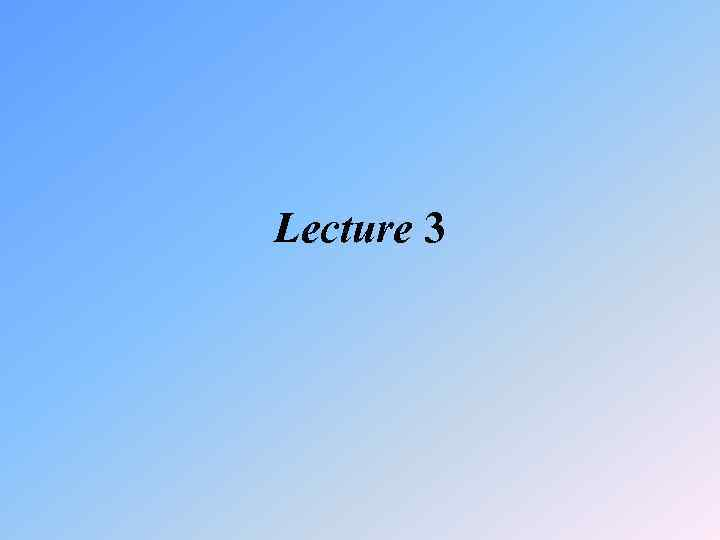Lecture 3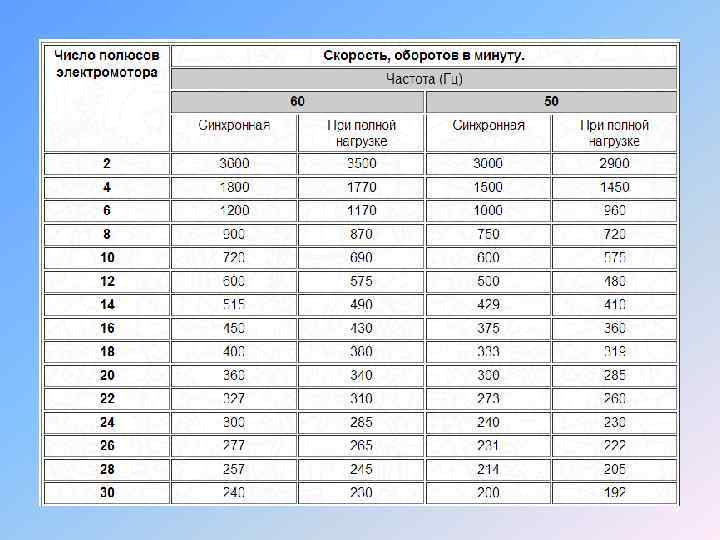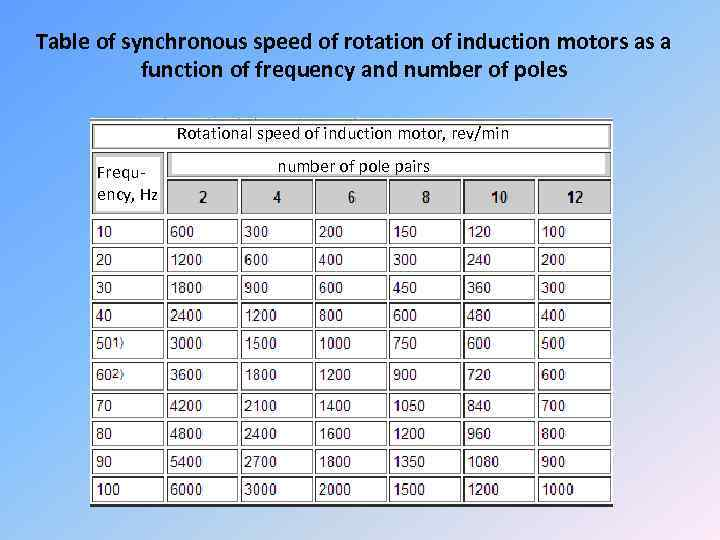Table of synchronous speed of rotation of induction motors as a function of frequency and number of poles Rotational speed of induction motor, rev/min Frequency, Hz number of pole pairs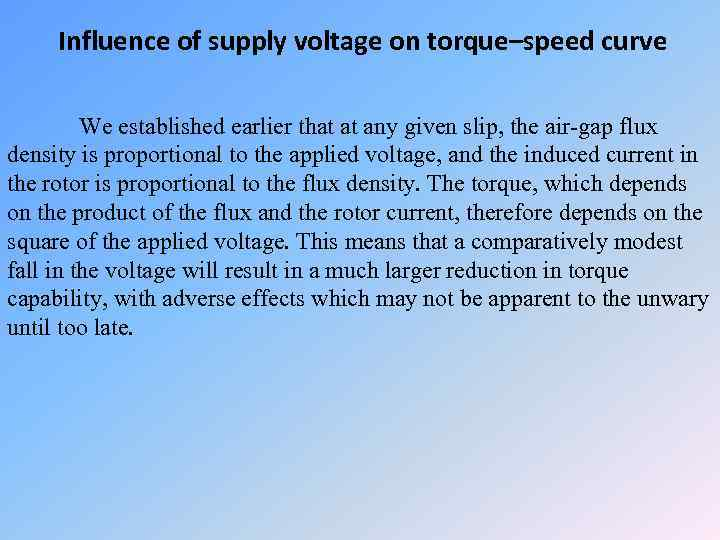Influence of supply voltage on torque–speed curve We established earlier that at any given slip, the air-gap flux density is proportional to the applied voltage, and the induced current in the rotor is proportional to the flux density. The torque, which depends on the product of the flux and the rotor current, therefore depends on the square of the applied voltage. This means that a comparatively modest fall in the voltage will result in a much larger reduction in torque capability, with adverse effects which may not be apparent to the unwary until too late.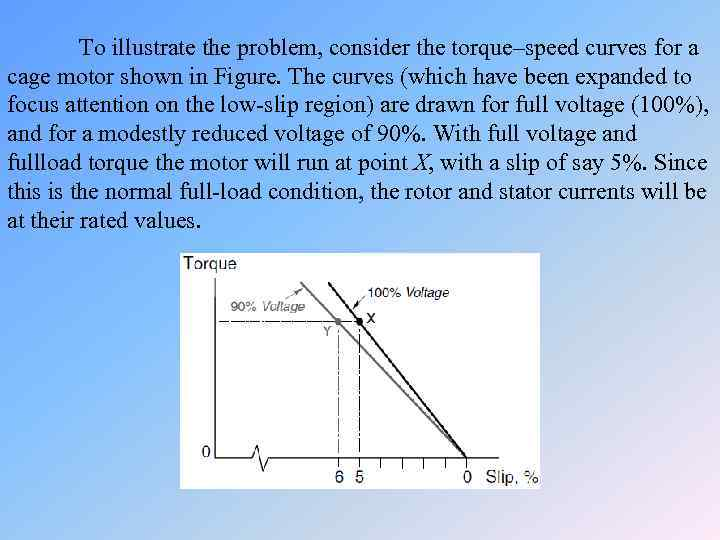To illustrate the problem, consider the torque–speed curves for a cage motor shown in Figure. The curves (which have been expanded to focus attention on the low-slip region) are drawn for full voltage (100%), and for a modestly reduced voltage of 90%. With full voltage and fullload torque the motor will run at point X, with a slip of say 5%. Since this is the normal full-load condition, the rotor and stator currents will be at their rated values.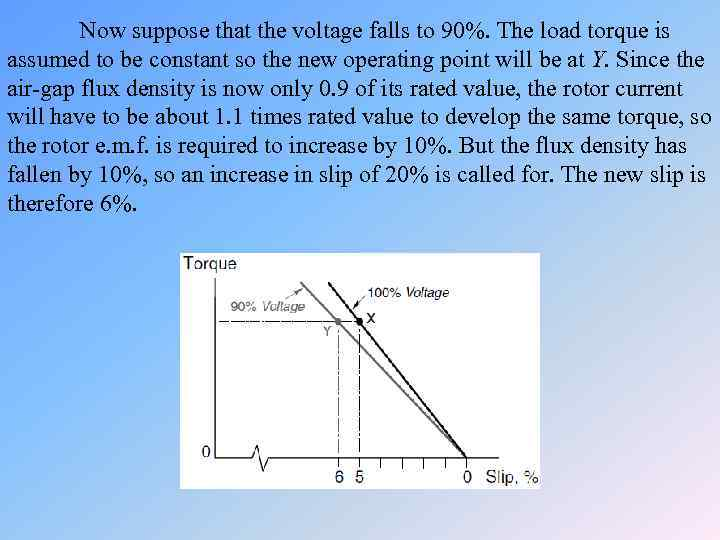Now suppose that the voltage falls to 90%. The load torque is assumed to be constant so the new operating point will be at Y. Since the air-gap flux density is now only 0. 9 of its rated value, the rotor current will have to be about 1. 1 times rated value to develop the same torque, so the rotor e. m. f. is required to increase by 10%. But the flux density has fallen by 10%, so an increase in slip of 20% is called for. The new slip is therefore 6%.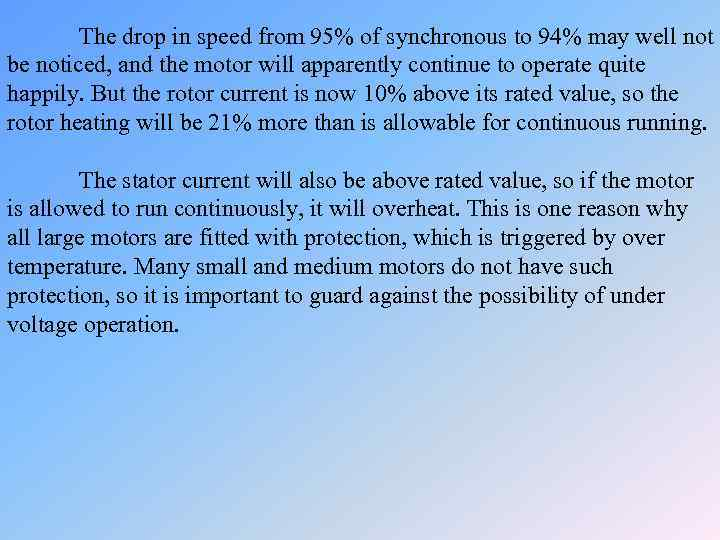The drop in speed from 95% of synchronous to 94% may well not be noticed, and the motor will apparently continue to operate quite happily. But the rotor current is now 10% above its rated value, so the rotor heating will be 21% more than is allowable for continuous running. The stator current will also be above rated value, so if the motor is allowed to run continuously, it will overheat. This is one reason why all large motors are fitted with protection, which is triggered by over temperature. Many small and medium motors do not have such protection, so it is important to guard against the possibility of under voltage operation.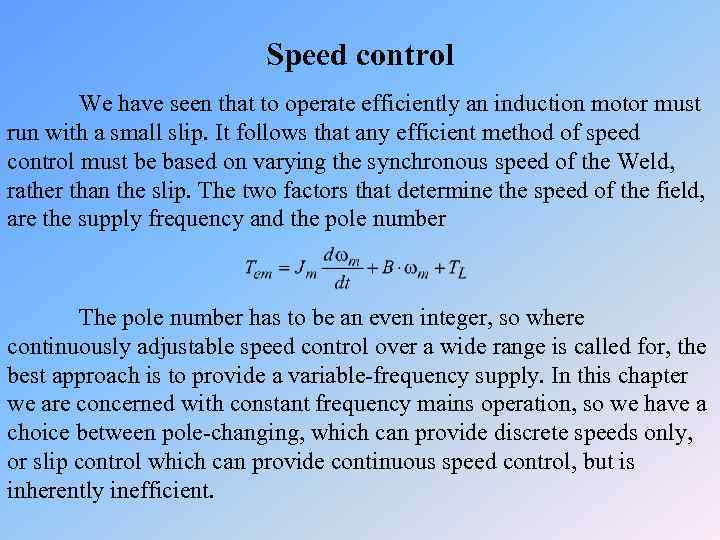Speed control We have seen that to operate efficiently an induction motor must run with a small slip. It follows that any efficient method of speed control must be based on varying the synchronous speed of the Weld, rather than the slip. The two factors that determine the speed of the field, are the supply frequency and the pole number The pole number has to be an even integer, so where continuously adjustable speed control over a wide range is called for, the best approach is to provide a variable-frequency supply. In this chapter we are concerned with constant frequency mains operation, so we have a choice between pole-changing, which can provide discrete speeds only, or slip control which can provide continuous speed control, but is inherently inefficient.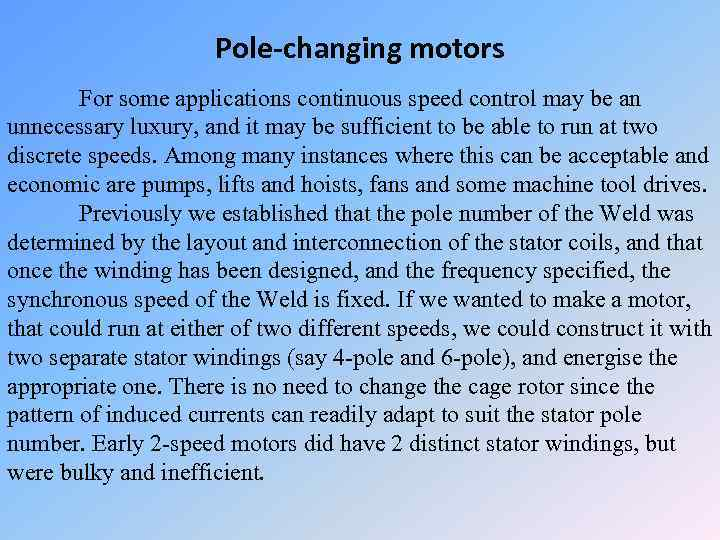Pole-changing motors For some applications continuous speed control may be an unnecessary luxury, and it may be sufficient to be able to run at two discrete speeds. Among many instances where this can be acceptable and economic are pumps, lifts and hoists, fans and some machine tool drives. Previously we established that the pole number of the Weld was determined by the layout and interconnection of the stator coils, and that once the winding has been designed, and the frequency specified, the synchronous speed of the Weld is fixed. If we wanted to make a motor, that could run at either of two different speeds, we could construct it with two separate stator windings (say 4 -pole and 6 -pole), and energise the appropriate one. There is no need to change the cage rotor since the pattern of induced currents can readily adapt to suit the stator pole number. Early 2 -speed motors did have 2 distinct stator windings, but were bulky and inefficient.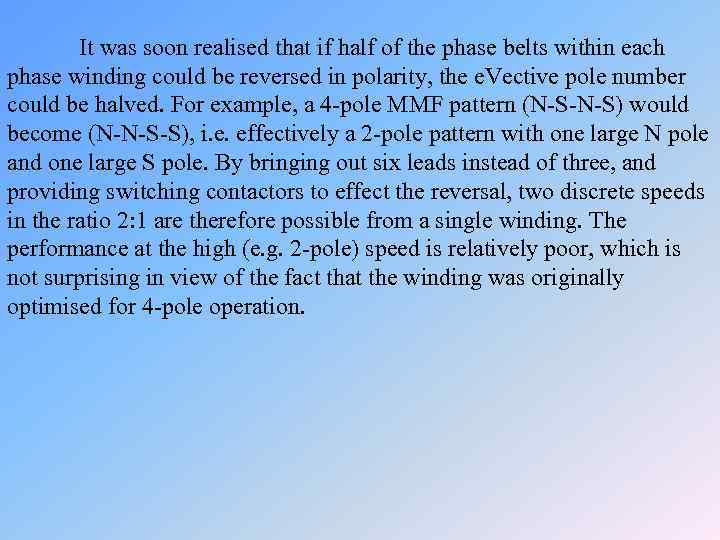It was soon realised that if half of the phase belts within each phase winding could be reversed in polarity, the e. Vective pole number could be halved. For example, a 4 -pole MMF pattern (N-S-N-S) would become (N-N-S-S), i. e. effectively a 2 -pole pattern with one large N pole and one large S pole. By bringing out six leads instead of three, and providing switching contactors to effect the reversal, two discrete speeds in the ratio 2: 1 are therefore possible from a single winding. The performance at the high (e. g. 2 -pole) speed is relatively poor, which is not surprising in view of the fact that the winding was originally optimised for 4 -pole operation.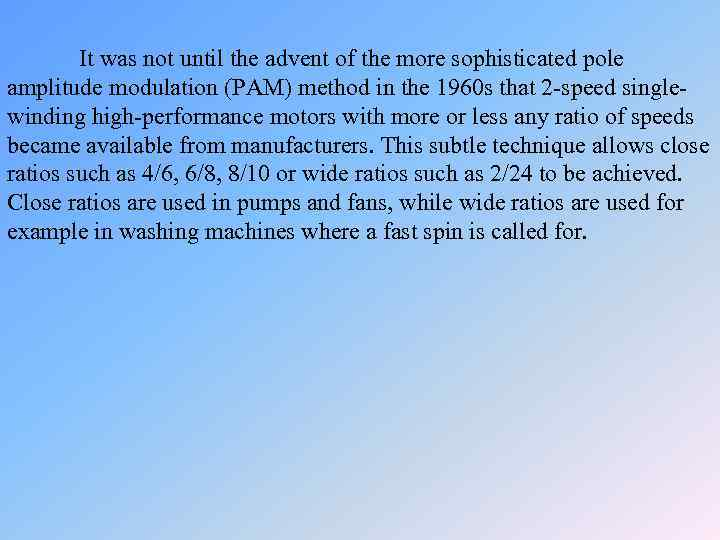It was not until the advent of the more sophisticated pole amplitude modulation (PAM) method in the 1960 s that 2 -speed singlewinding high-performance motors with more or less any ratio of speeds became available from manufacturers. This subtle technique allows close ratios such as 4/6, 6/8, 8/10 or wide ratios such as 2/24 to be achieved. Close ratios are used in pumps and fans, while wide ratios are used for example in washing machines where a fast spin is called for.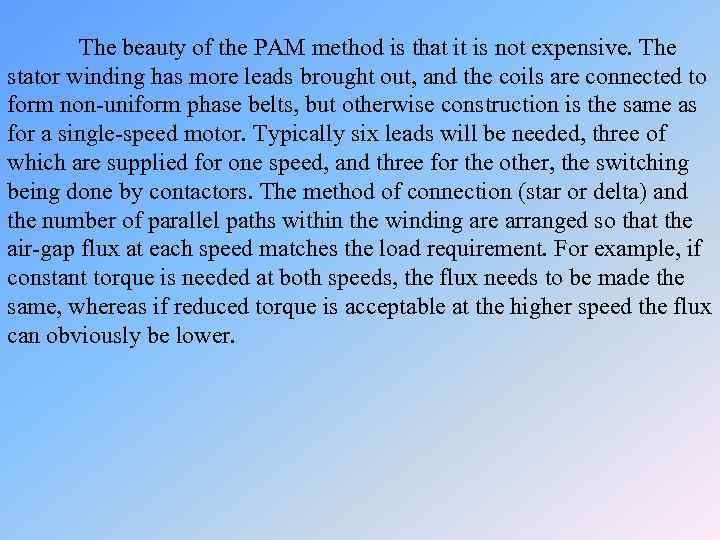The beauty of the PAM method is that it is not expensive. The stator winding has more leads brought out, and the coils are connected to form non-uniform phase belts, but otherwise construction is the same as for a single-speed motor. Typically six leads will be needed, three of which are supplied for one speed, and three for the other, the switching being done by contactors. The method of connection (star or delta) and the number of parallel paths within the winding are arranged so that the air-gap flux at each speed matches the load requirement. For example, if constant torque is needed at both speeds, the flux needs to be made the same, whereas if reduced torque is acceptable at the higher speed the flux can obviously be lower.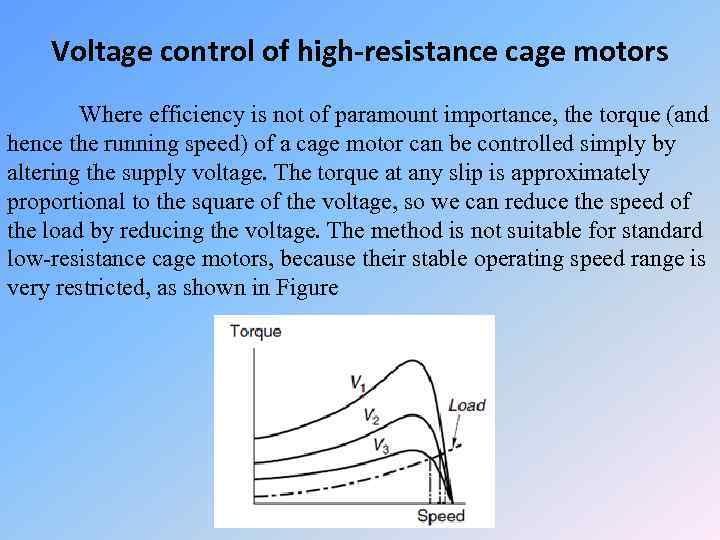Voltage control of high-resistance cage motors Where efficiency is not of paramount importance, the torque (and hence the running speed) of a cage motor can be controlled simply by altering the supply voltage. The torque at any slip is approximately proportional to the square of the voltage, so we can reduce the speed of the load by reducing the voltage. The method is not suitable for standard low-resistance cage motors, because their stable operating speed range is very restricted, as shown in Figure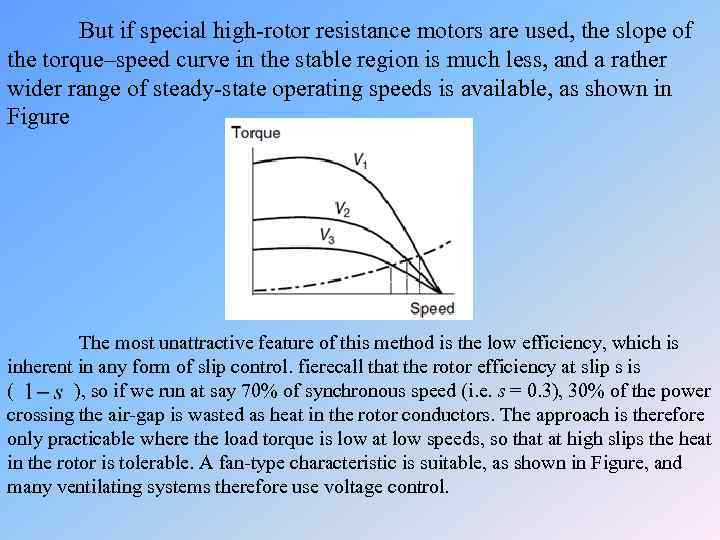But if special high-rotor resistance motors are used, the slope of the torque–speed curve in the stable region is much less, and a rather wider range of steady-state operating speeds is available, as shown in Figure The most unattractive feature of this method is the low efficiency, which is inherent in any form of slip control. fierecall that the rotor efficiency at slip s is ( ), so if we run at say 70% of synchronous speed (i. e. s = 0. 3), 30% of the power crossing the air-gap is wasted as heat in the rotor conductors. The approach is therefore only practicable where the load torque is low at low speeds, so that at high slips the heat in the rotor is tolerable. A fan-type characteristic is suitable, as shown in Figure, and many ventilating systems therefore use voltage control.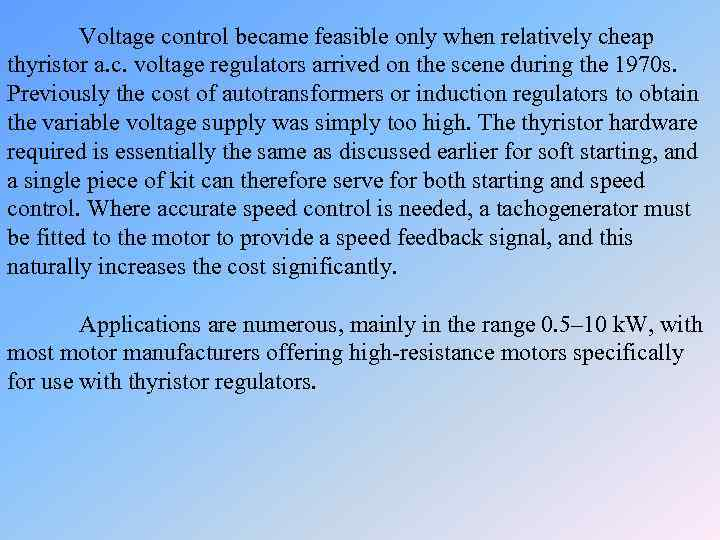Voltage control became feasible only when relatively cheap thyristor a. c. voltage regulators arrived on the scene during the 1970 s. Previously the cost of autotransformers or induction regulators to obtain the variable voltage supply was simply too high. The thyristor hardware required is essentially the same as discussed earlier for soft starting, and a single piece of kit can therefore serve for both starting and speed control. Where accurate speed control is needed, a tachogenerator must be fitted to the motor to provide a speed feedback signal, and this naturally increases the cost significantly. Applications are numerous, mainly in the range 0. 5– 10 k. W, with most motor manufacturers offering high-resistance motors specifically for use with thyristor regulators.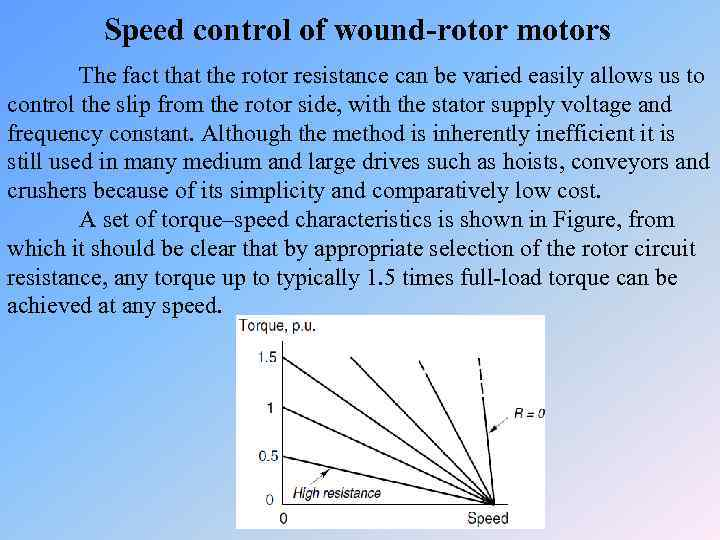Speed control of wound-rotor motors The fact that the rotor resistance can be varied easily allows us to control the slip from the rotor side, with the stator supply voltage and frequency constant. Although the method is inherently inefficient it is still used in many medium and large drives such as hoists, conveyors and crushers because of its simplicity and comparatively low cost. A set of torque–speed characteristics is shown in Figure, from which it should be clear that by appropriate selection of the rotor circuit resistance, any torque up to typically 1. 5 times full-load torque can be achieved at any speed.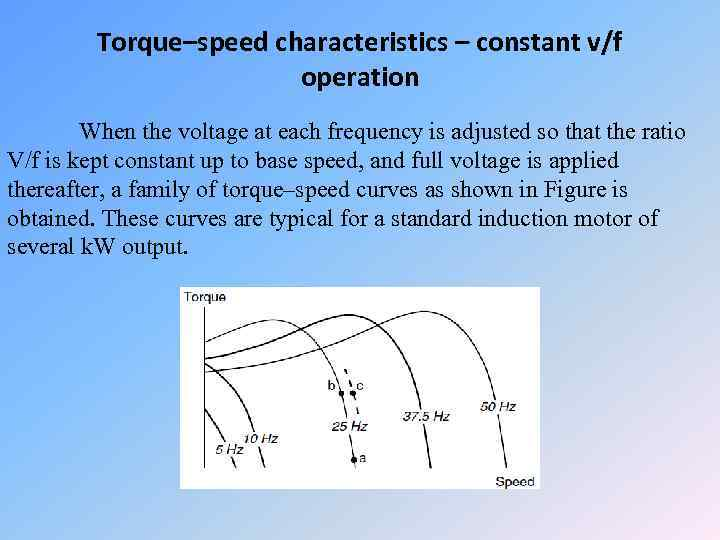Torque–speed characteristics – constant v/f operation When the voltage at each frequency is adjusted so that the ratio V/f is kept constant up to base speed, and full voltage is applied thereafter, a family of torque–speed curves as shown in Figure is obtained. These curves are typical for a standard induction motor of several k. W output.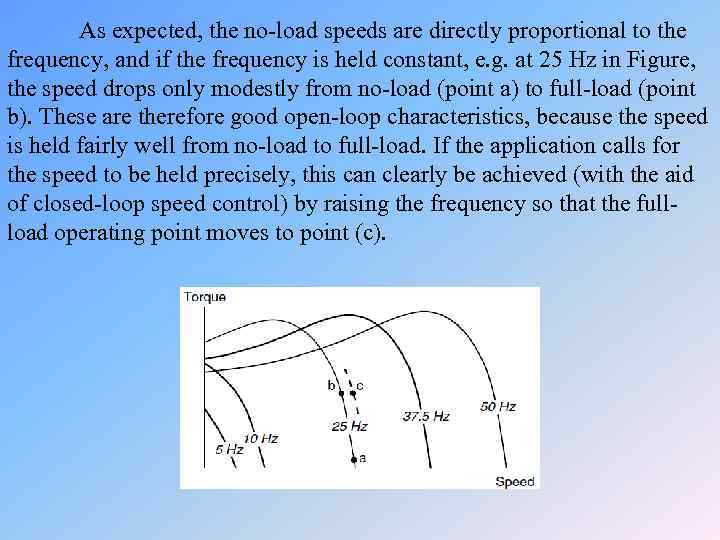As expected, the no-load speeds are directly proportional to the frequency, and if the frequency is held constant, e. g. at 25 Hz in Figure, the speed drops only modestly from no-load (point a) to full-load (point b). These are therefore good open-loop characteristics, because the speed is held fairly well from no-load to full-load. If the application calls for the speed to be held precisely, this can clearly be achieved (with the aid of closed-loop speed control) by raising the frequency so that the fullload operating point moves to point (c).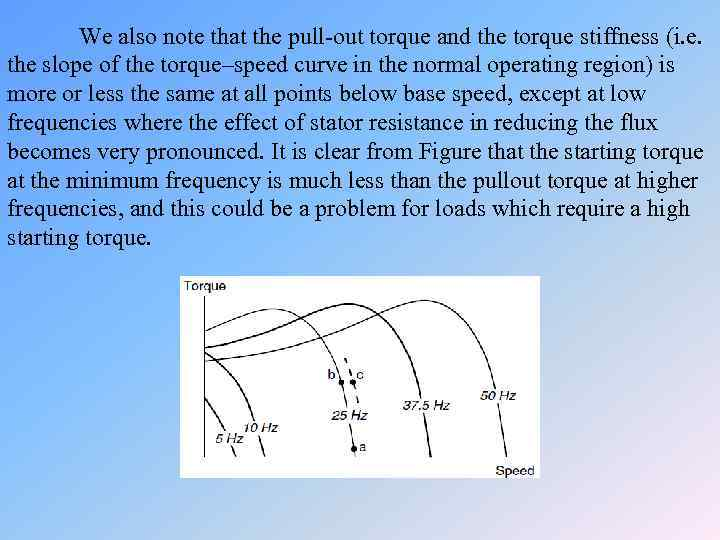We also note that the pull-out torque and the torque stiffness (i. e. the slope of the torque–speed curve in the normal operating region) is more or less the same at all points below base speed, except at low frequencies where the effect of stator resistance in reducing the flux becomes very pronounced. It is clear from Figure that the starting torque at the minimum frequency is much less than the pullout torque at higher frequencies, and this could be a problem for loads which require a high starting torque.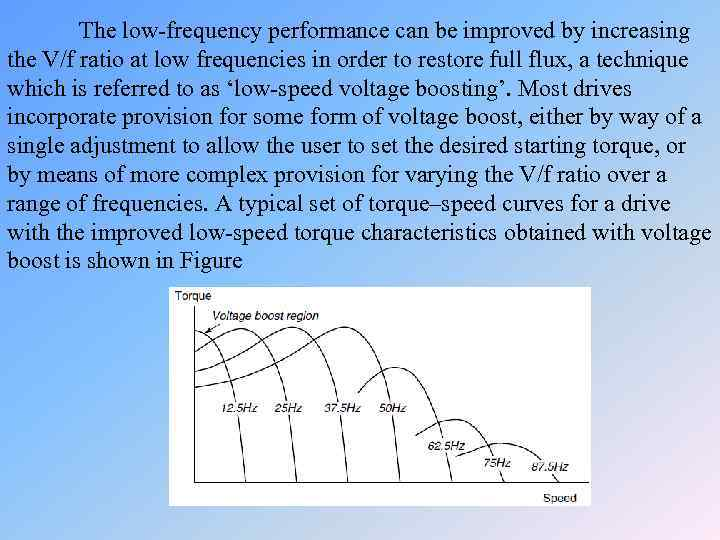The low-frequency performance can be improved by increasing the V/f ratio at low frequencies in order to restore full flux, a technique which is referred to as ‘low-speed voltage boosting’. Most drives incorporate provision for some form of voltage boost, either by way of a single adjustment to allow the user to set the desired starting torque, or by means of more complex provision for varying the V/f ratio over a range of frequencies. A typical set of torque–speed curves for a drive with the improved low-speed torque characteristics obtained with voltage boost is shown in Figure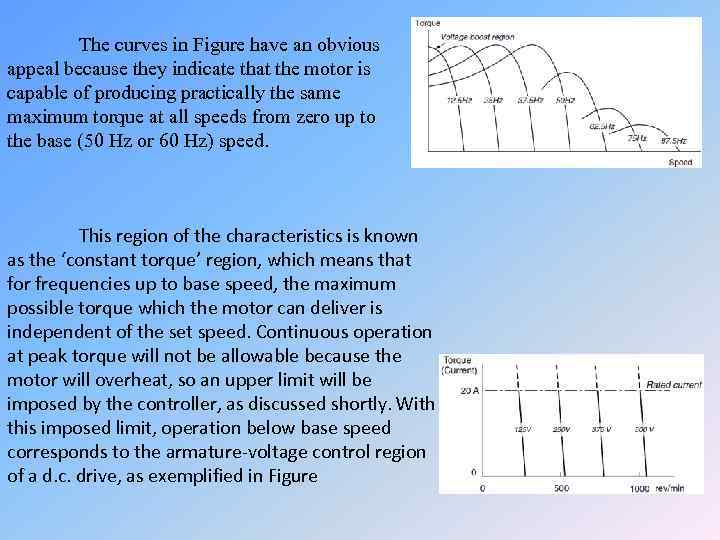The curves in Figure have an obvious appeal because they indicate that the motor is capable of producing practically the same maximum torque at all speeds from zero up to the base (50 Hz or 60 Hz) speed. This region of the characteristics is known as the ‘constant torque’ region, which means that for frequencies up to base speed, the maximum possible torque which the motor can deliver is independent of the set speed. Continuous operation at peak torque will not be allowable because the motor will overheat, so an upper limit will be imposed by the controller, as discussed shortly. With this imposed limit, operation below base speed corresponds to the armature-voltage control region of a d. c. drive, as exemplified in Figure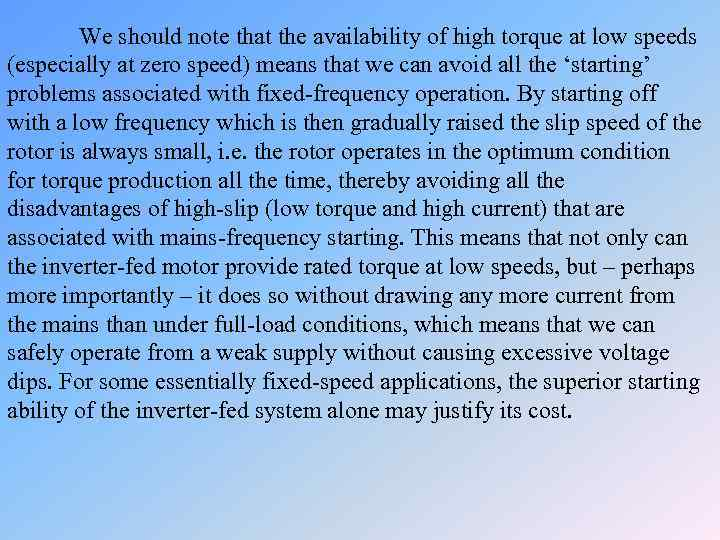We should note that the availability of high torque at low speeds (especially at zero speed) means that we can avoid all the ‘starting’ problems associated with fixed-frequency operation. By starting off with a low frequency which is then gradually raised the slip speed of the rotor is always small, i. e. the rotor operates in the optimum condition for torque production all the time, thereby avoiding all the disadvantages of high-slip (low torque and high current) that are associated with mains-frequency starting. This means that not only can the inverter-fed motor provide rated torque at low speeds, but – perhaps more importantly – it does so without drawing any more current from the mains than under full-load conditions, which means that we can safely operate from a weak supply without causing excessive voltage dips. For some essentially fixed-speed applications, the superior starting ability of the inverter-fed system alone may justify its cost.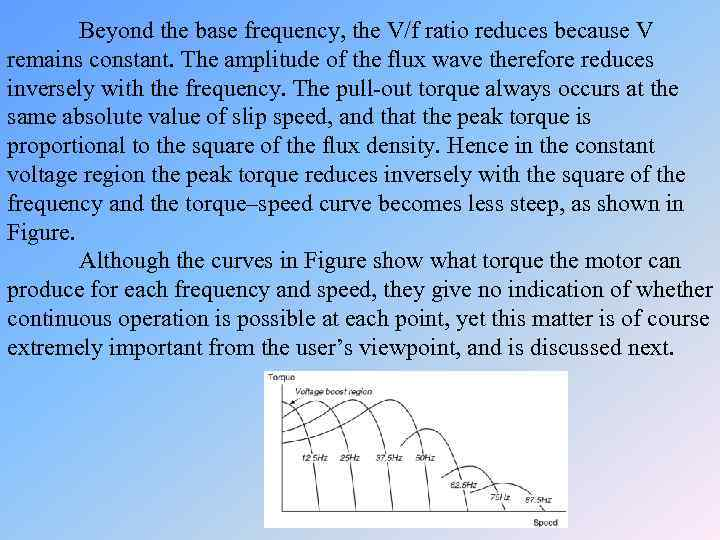Beyond the base frequency, the V/f ratio reduces because V remains constant. The amplitude of the flux wave therefore reduces inversely with the frequency. The pull-out torque always occurs at the same absolute value of slip speed, and that the peak torque is proportional to the square of the flux density. Hence in the constant voltage region the peak torque reduces inversely with the square of the frequency and the torque–speed curve becomes less steep, as shown in Figure. Although the curves in Figure show what torque the motor can produce for each frequency and speed, they give no indication of whether continuous operation is possible at each point, yet this matter is of course extremely important from the user’s viewpoint, and is discussed next.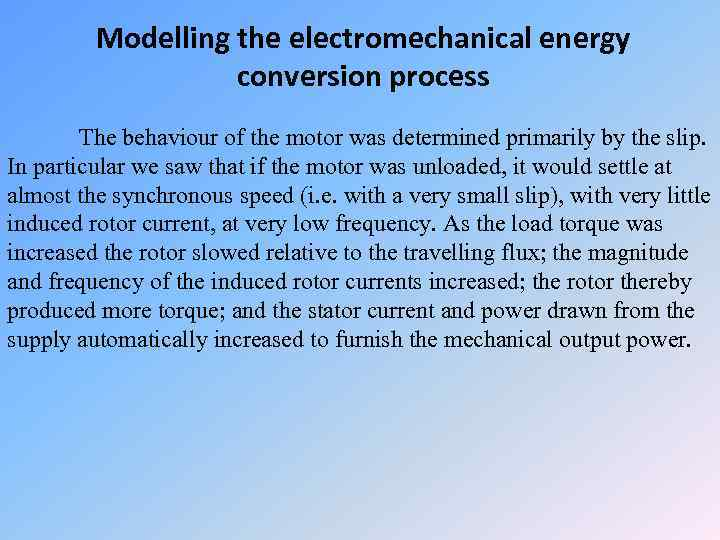Modelling the electromechanical energy conversion process The behaviour of the motor was determined primarily by the slip. In particular we saw that if the motor was unloaded, it would settle at almost the synchronous speed (i. e. with a very small slip), with very little induced rotor current, at very low frequency. As the load torque was increased the rotor slowed relative to the travelling flux; the magnitude and frequency of the induced rotor currents increased; the rotor thereby produced more torque; and the stator current and power drawn from the supply automatically increased to furnish the mechanical output power.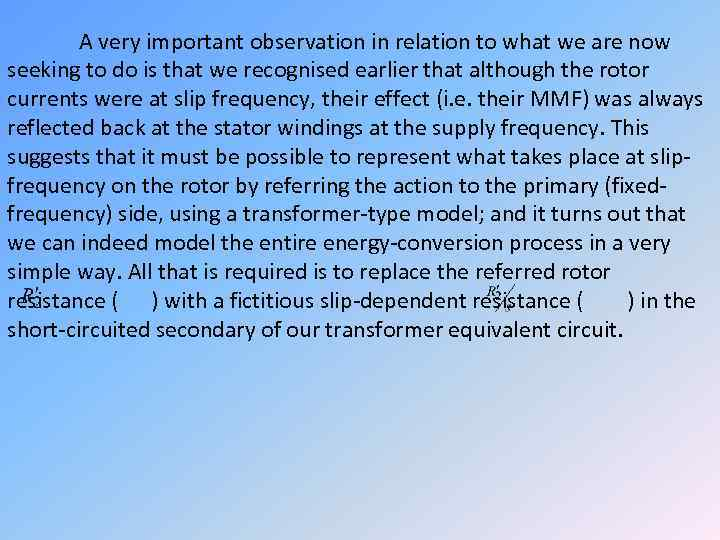A very important observation in relation to what we are now seeking to do is that we recognised earlier that although the rotor currents were at slip frequency, their effect (i. e. their MMF) was always reflected back at the stator windings at the supply frequency. This suggests that it must be possible to represent what takes place at slipfrequency on the rotor by referring the action to the primary (fixedfrequency) side, using a transformer-type model; and it turns out that we can indeed model the entire energy-conversion process in a very simple way. All that is required is to replace the referred rotor resistance ( ) with a fictitious slip-dependent resistance ( ) in the short-circuited secondary of our transformer equivalent circuit.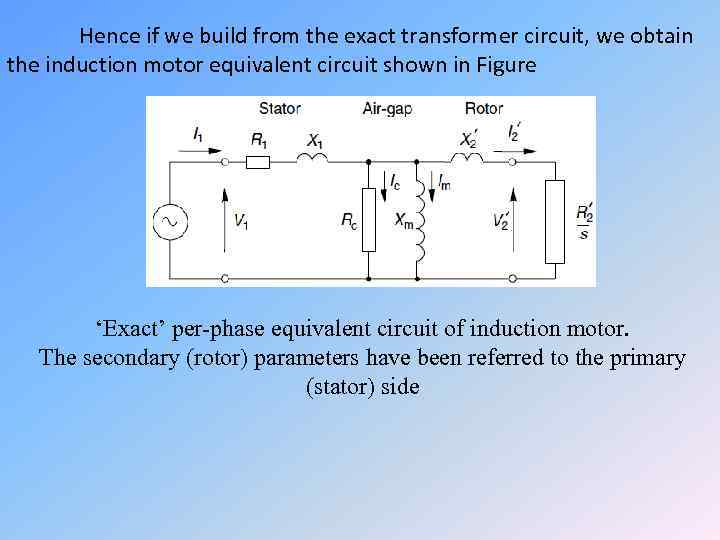Hence if we build from the exact transformer circuit, we obtain the induction motor equivalent circuit shown in Figure ‘Exact’ per-phase equivalent circuit of induction motor. The secondary (rotor) parameters have been referred to the primary (stator) side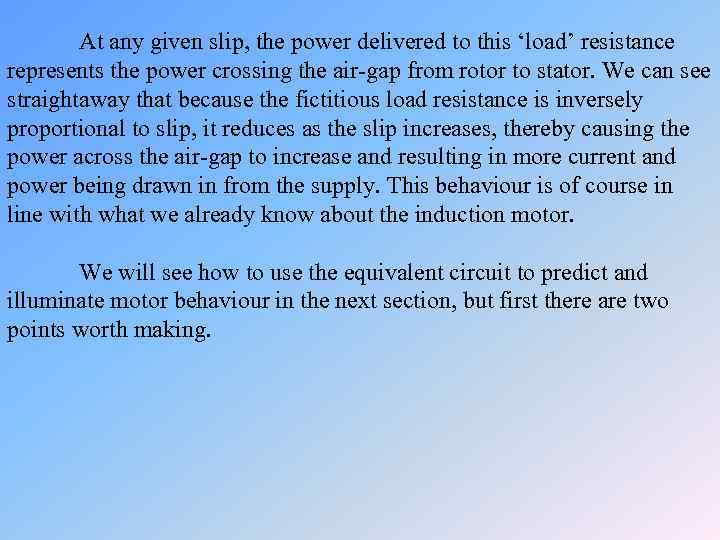At any given slip, the power delivered to this ‘load’ resistance represents the power crossing the air-gap from rotor to stator. We can see straightaway that because the fictitious load resistance is inversely proportional to slip, it reduces as the slip increases, thereby causing the power across the air-gap to increase and resulting in more current and power being drawn in from the supply. This behaviour is of course in line with what we already know about the induction motor. We will see how to use the equivalent circuit to predict and illuminate motor behaviour in the next section, but first there are two points worth making.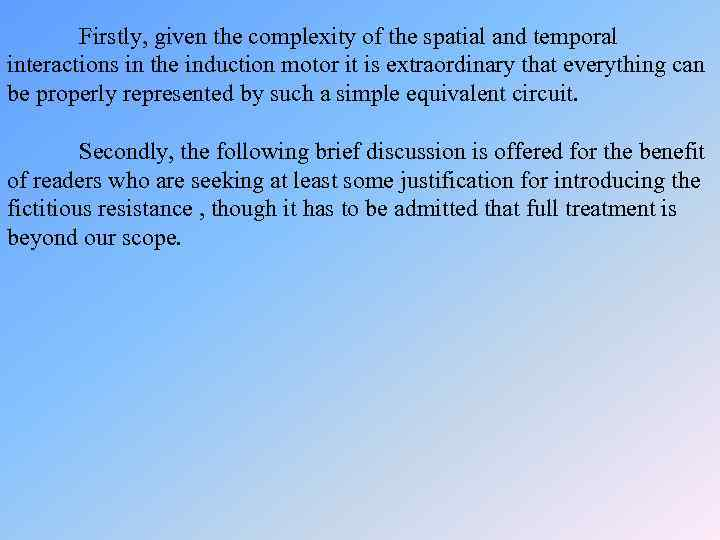Firstly, given the complexity of the spatial and temporal interactions in the induction motor it is extraordinary that everything can be properly represented by such a simple equivalent circuit. Secondly, the following brief discussion is offered for the benefit of readers who are seeking at least some justification for introducing the fictitious resistance , though it has to be admitted that full treatment is beyond our scope.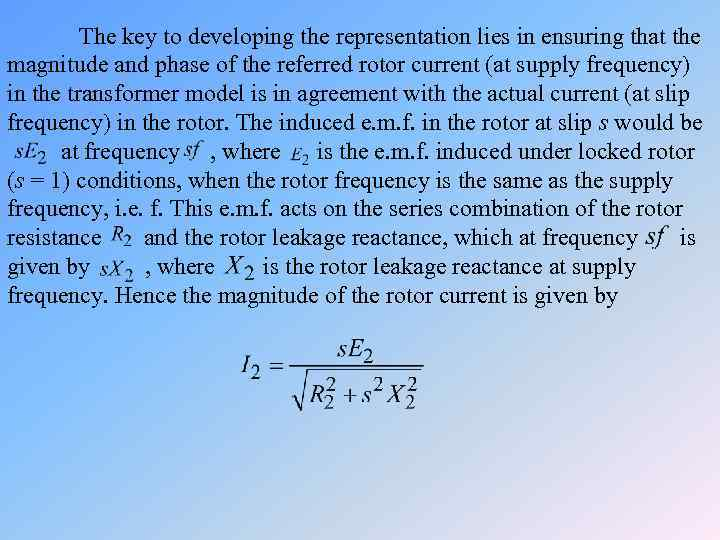The key to developing the representation lies in ensuring that the magnitude and phase of the referred rotor current (at supply frequency) in the transformer model is in agreement with the actual current (at slip frequency) in the rotor. The induced e. m. f. in the rotor at slip s would be at frequency , where is the e. m. f. induced under locked rotor (s = 1) conditions, when the rotor frequency is the same as the supply frequency, i. e. f. This e. m. f. acts on the series combination of the rotor resistance and the rotor leakage reactance, which at frequency is given by , where is the rotor leakage reactance at supply frequency. Hence the magnitude of the rotor current is given by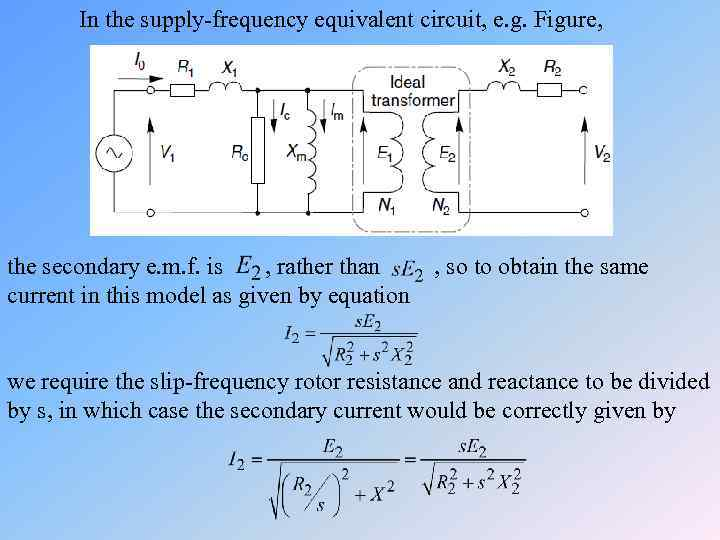In the supply-frequency equivalent circuit, e. g. Figure, the secondary e. m. f. is , rather than , so to obtain the same current in this model as given by equation we require the slip-frequency rotor resistance and reactance to be divided by s, in which case the secondary current would be correctly given by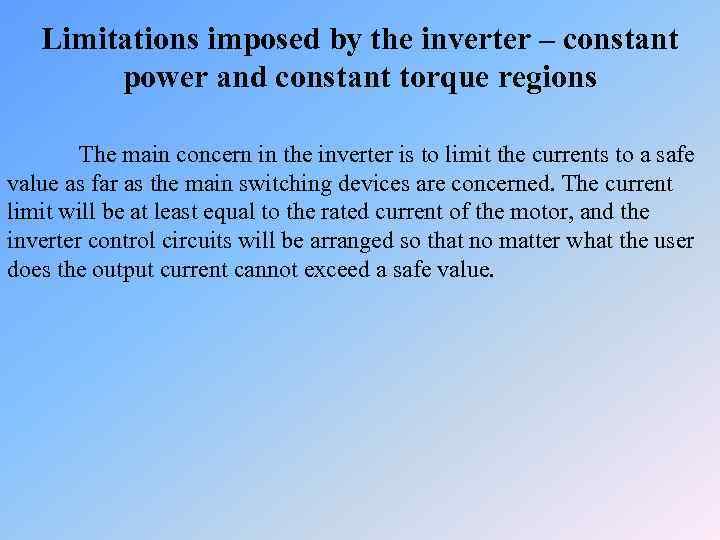Limitations imposed by the inverter – constant power and constant torque regions The main concern in the inverter is to limit the currents to a safe value as far as the main switching devices are concerned. The current limit will be at least equal to the rated current of the motor, and the inverter control circuits will be arranged so that no matter what the user does the output current cannot exceed a safe value.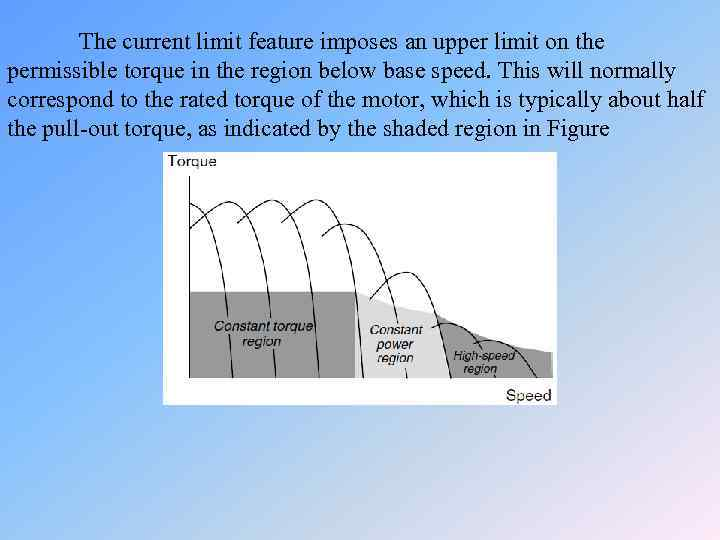The current limit feature imposes an upper limit on the permissible torque in the region below base speed. This will normally correspond to the rated torque of the motor, which is typically about half the pull-out torque, as indicated by the shaded region in Figure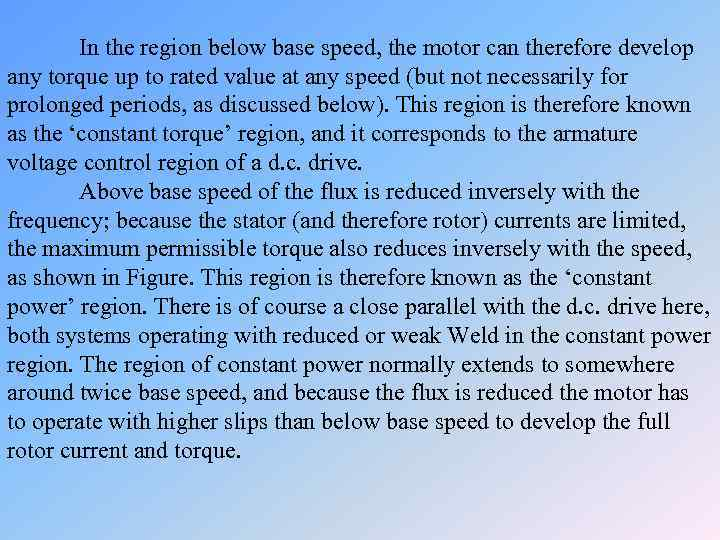In the region below base speed, the motor can therefore develop any torque up to rated value at any speed (but not necessarily for prolonged periods, as discussed below). This region is therefore known as the ‘constant torque’ region, and it corresponds to the armature voltage control region of a d. c. drive. Above base speed of the flux is reduced inversely with the frequency; because the stator (and therefore rotor) currents are limited, the maximum permissible torque also reduces inversely with the speed, as shown in Figure. This region is therefore known as the ‘constant power’ region. There is of course a close parallel with the d. c. drive here, both systems operating with reduced or weak Weld in the constant power region. The region of constant power normally extends to somewhere around twice base speed, and because the flux is reduced the motor has to operate with higher slips than below base speed to develop the full rotor current and torque.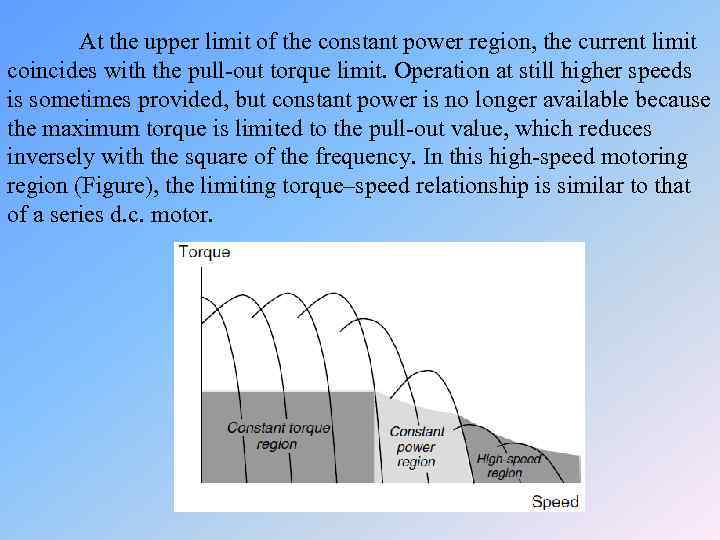At the upper limit of the constant power region, the current limit coincides with the pull-out torque limit. Operation at still higher speeds is sometimes provided, but constant power is no longer available because the maximum torque is limited to the pull-out value, which reduces inversely with the square of the frequency. In this high-speed motoring region (Figure), the limiting torque–speed relationship is similar to that of a series d. c. motor.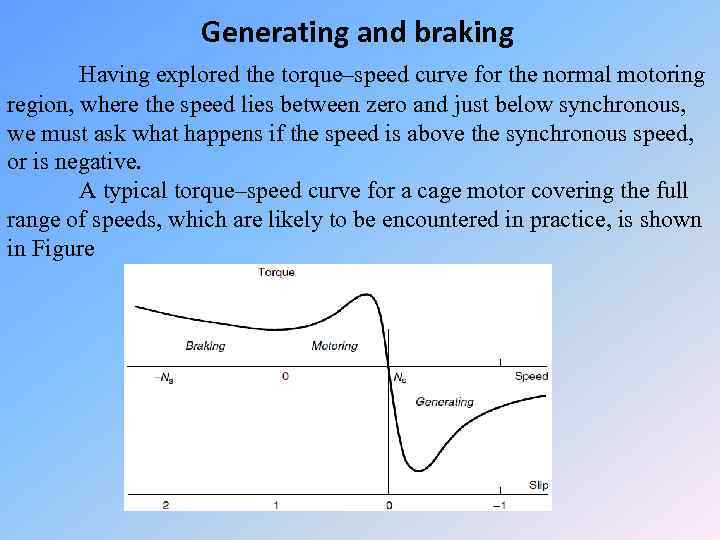Generating and braking Having explored the torque–speed curve for the normal motoring region, where the speed lies between zero and just below synchronous, we must ask what happens if the speed is above the synchronous speed, or is negative. A typical torque–speed curve for a cage motor covering the full range of speeds, which are likely to be encountered in practice, is shown in Figure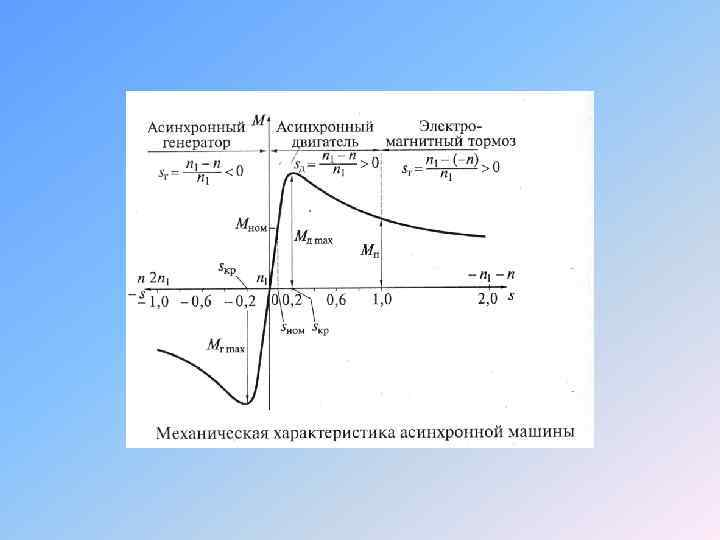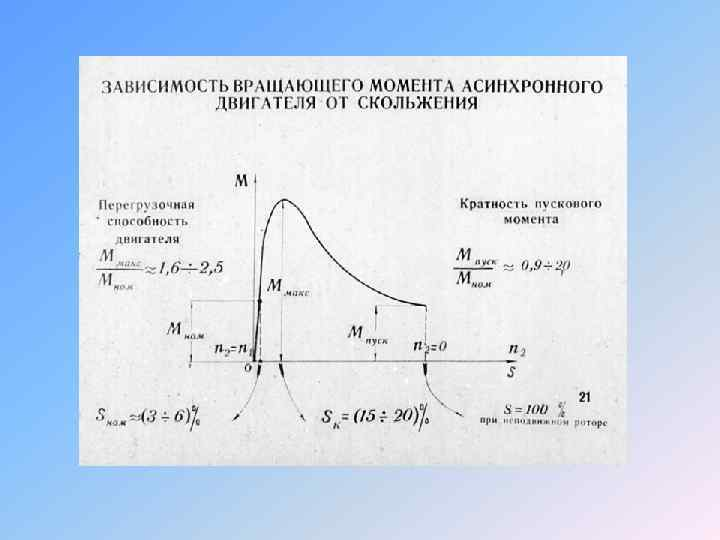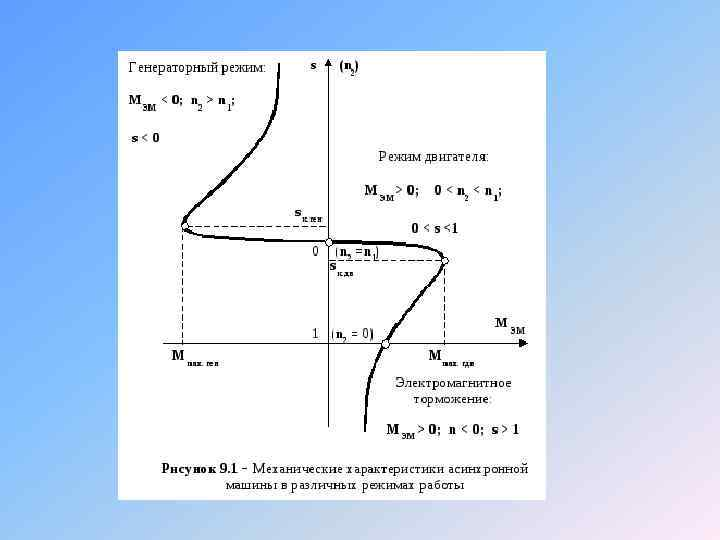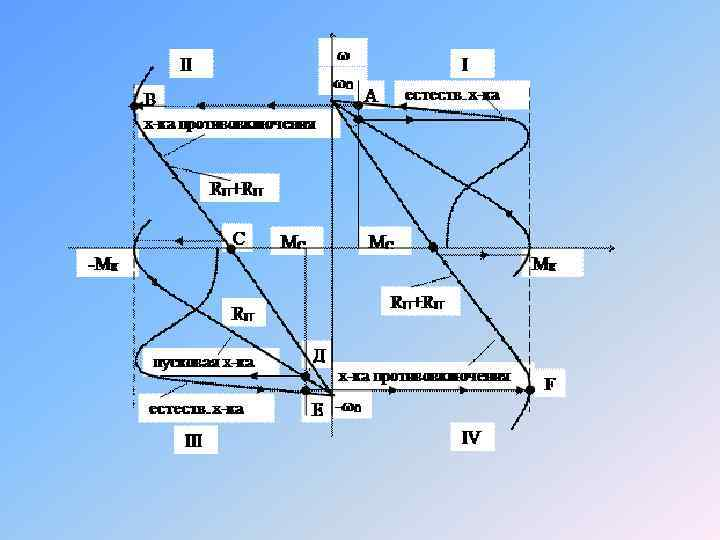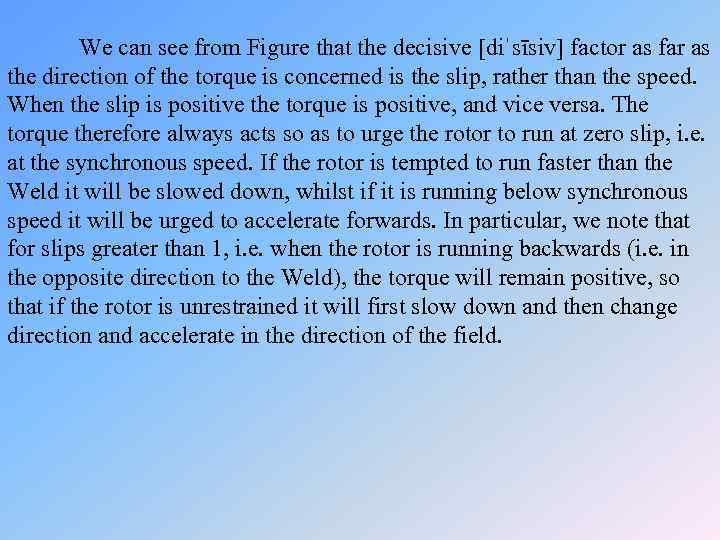We can see from Figure that the decisive [diˈsīsiv] factor as far as the direction of the torque is concerned is the slip, rather than the speed. When the slip is positive the torque is positive, and vice versa. The torque therefore always acts so as to urge the rotor to run at zero slip, i. e. at the synchronous speed. If the rotor is tempted to run faster than the Weld it will be slowed down, whilst if it is running below synchronous speed it will be urged to accelerate forwards. In particular, we note that for slips greater than 1, i. e. when the rotor is running backwards (i. e. in the opposite direction to the Weld), the torque will remain positive, so that if the rotor is unrestrained it will first slow down and then change direction and accelerate in the direction of the field.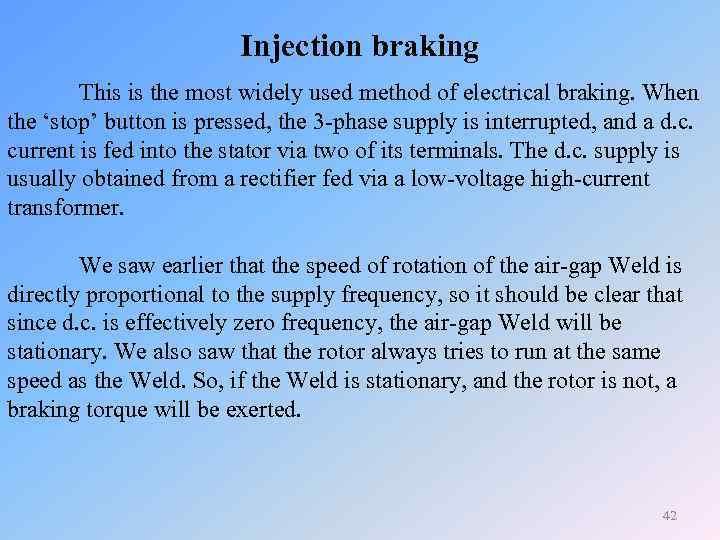Injection braking This is the most widely used method of electrical braking. When the ‘stop’ button is pressed, the 3 -phase supply is interrupted, and a d. c. current is fed into the stator via two of its terminals. The d. c. supply is usually obtained from a rectifier fed via a low-voltage high-current transformer. We saw earlier that the speed of rotation of the air-gap Weld is directly proportional to the supply frequency, so it should be clear that since d. c. is effectively zero frequency, the air-gap Weld will be stationary. We also saw that the rotor always tries to run at the same speed as the Weld. So, if the Weld is stationary, and the rotor is not, a braking torque will be exerted. 42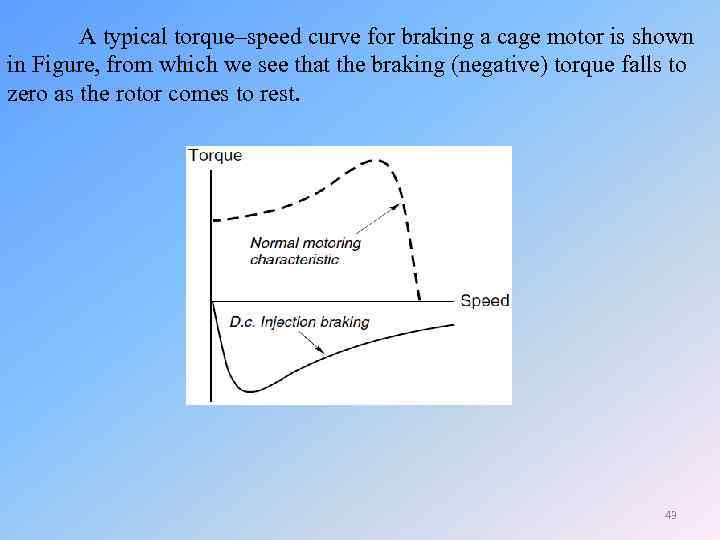A typical torque–speed curve for braking a cage motor is shown in Figure, from which we see that the braking (negative) torque falls to zero as the rotor comes to rest. 43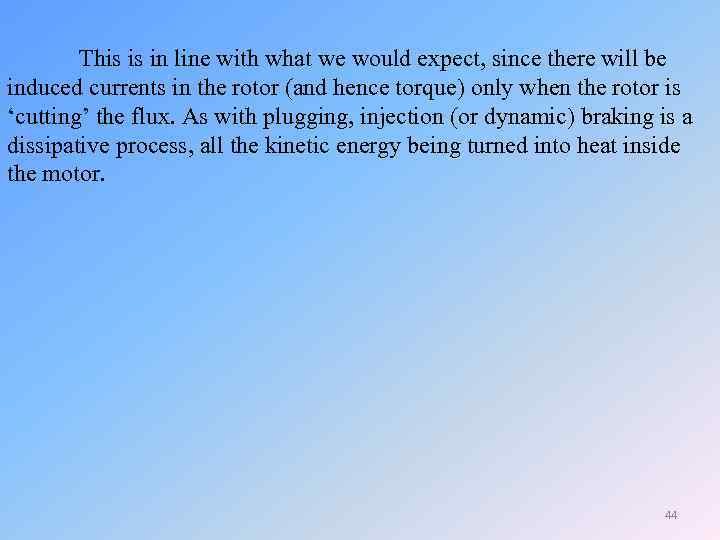This is in line with what we would expect, since there will be induced currents in the rotor (and hence torque) only when the rotor is ‘cutting’ the flux. As with plugging, injection (or dynamic) braking is a dissipative process, all the kinetic energy being turned into heat inside the motor. 44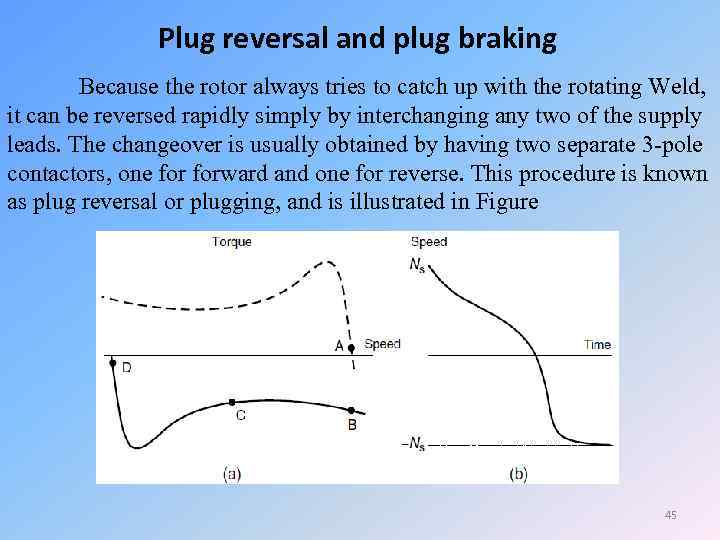Plug reversal and plug braking Because the rotor always tries to catch up with the rotating Weld, it can be reversed rapidly simply by interchanging any two of the supply leads. The changeover is usually obtained by having two separate 3 -pole contactors, one forward and one for reverse. This procedure is known as plug reversal or plugging, and is illustrated in Figure 45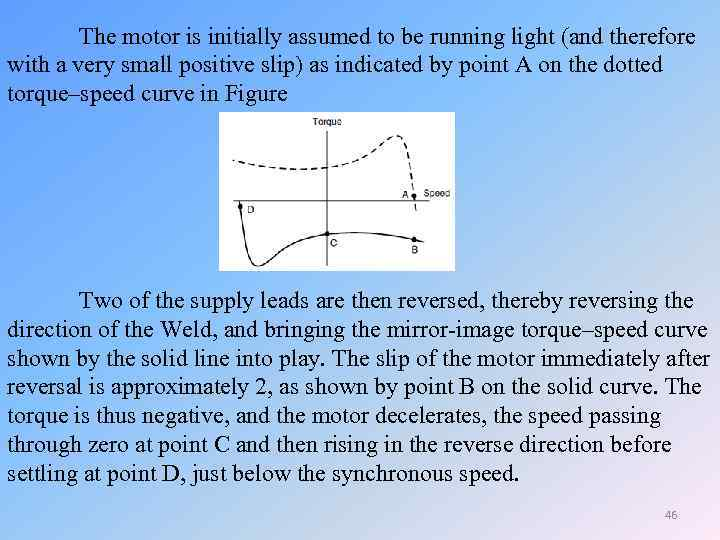The motor is initially assumed to be running light (and therefore with a very small positive slip) as indicated by point A on the dotted torque–speed curve in Figure Two of the supply leads are then reversed, thereby reversing the direction of the Weld, and bringing the mirror-image torque–speed curve shown by the solid line into play. The slip of the motor immediately after reversal is approximately 2, as shown by point B on the solid curve. The torque is thus negative, and the motor decelerates, the speed passing through zero at point C and then rising in the reverse direction before settling at point D, just below the synchronous speed. 46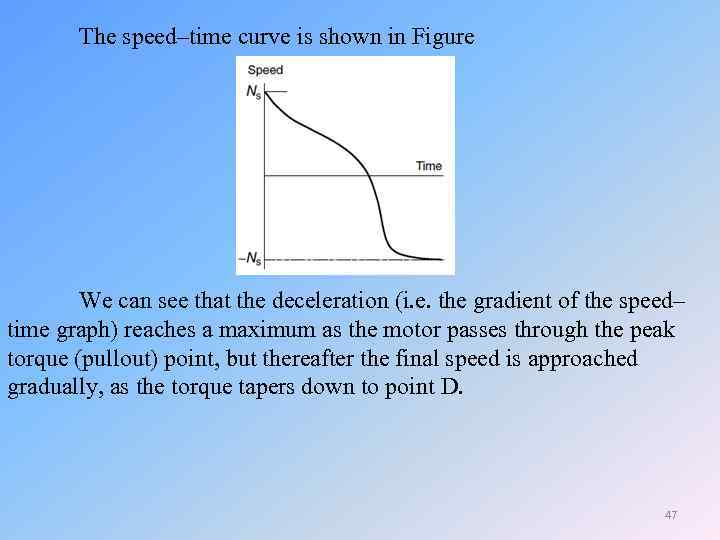The speed–time curve is shown in Figure We can see that the deceleration (i. e. the gradient of the speed– time graph) reaches a maximum as the motor passes through the peak torque (pullout) point, but thereafter the final speed is approached gradually, as the torque tapers down to point D. 47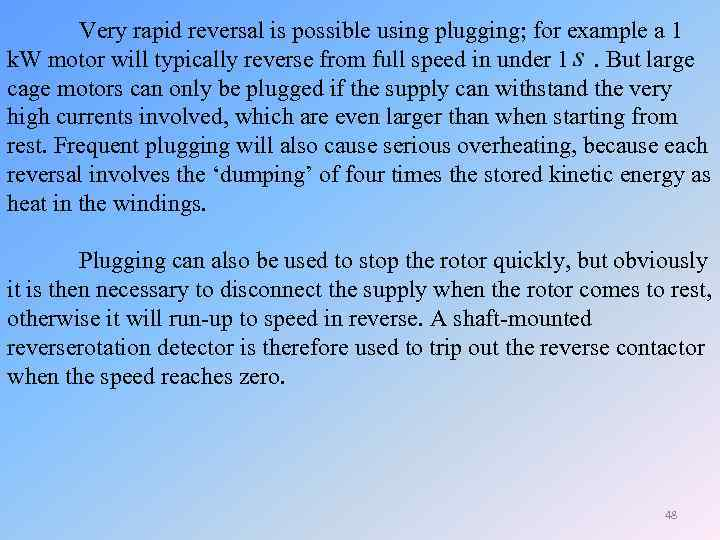Very rapid reversal is possible using plugging; for example a 1 k. W motor will typically reverse from full speed in under 1 . But large cage motors can only be plugged if the supply can withstand the very high currents involved, which are even larger than when starting from rest. Frequent plugging will also cause serious overheating, because each reversal involves the ‘dumping’ of four times the stored kinetic energy as heat in the windings. Plugging can also be used to stop the rotor quickly, but obviously it is then necessary to disconnect the supply when the rotor comes to rest, otherwise it will run-up to speed in reverse. A shaft-mounted reverserotation detector is therefore used to trip out the reverse contactor when the speed reaches zero. 48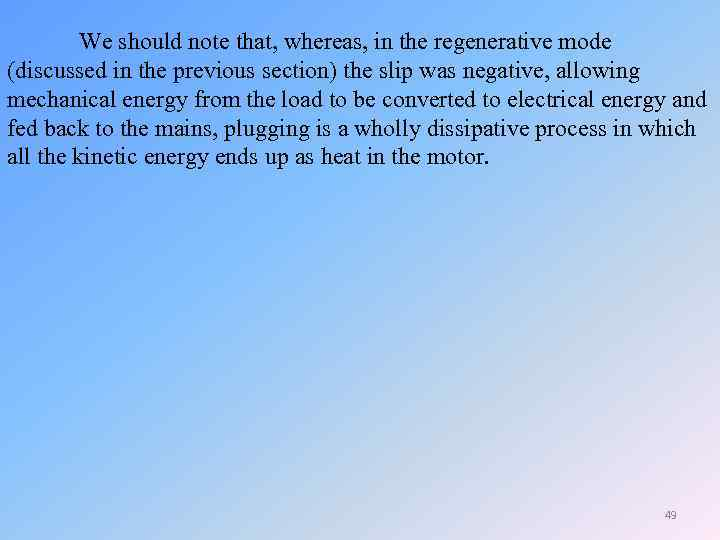We should note that, whereas, in the regenerative mode (discussed in the previous section) the slip was negative, allowing mechanical energy from the load to be converted to electrical energy and fed back to the mains, plugging is a wholly dissipative process in which all the kinetic energy ends up as heat in the motor. 49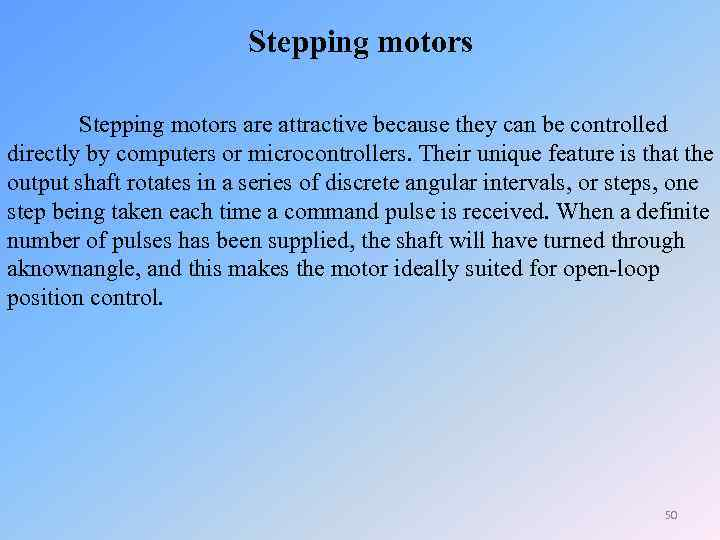Stepping motors are attractive because they can be controlled directly by computers or microcontrollers. Their unique feature is that the output shaft rotates in a series of discrete angular intervals, or steps, one step being taken each time a command pulse is received. When a definite number of pulses has been supplied, the shaft will have turned through aknownangle, and this makes the motor ideally suited for open-loop position control. 50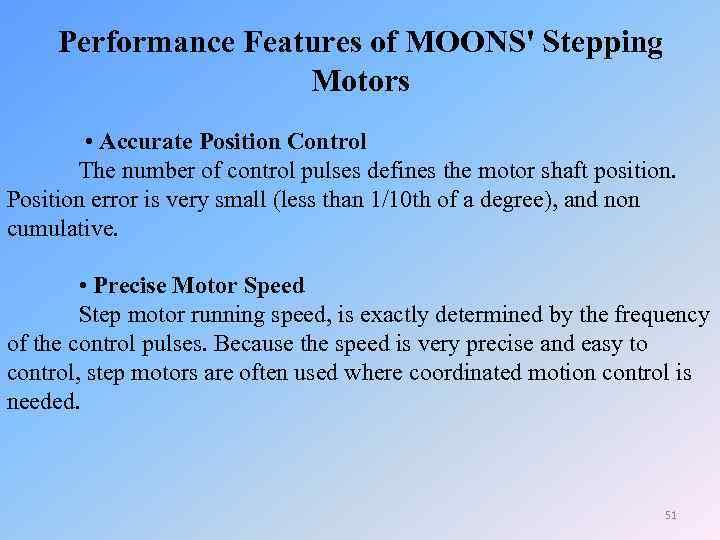Performance Features of MOONS' Stepping Motors • Accurate Position Control The number of control pulses defines the motor shaft position. Position error is very small (less than 1/10 th of a degree), and non cumulative. • Precise Motor Speed Step motor running speed, is exactly determined by the frequency of the control pulses. Because the speed is very precise and easy to control, step motors are often used where coordinated motion control is needed. 51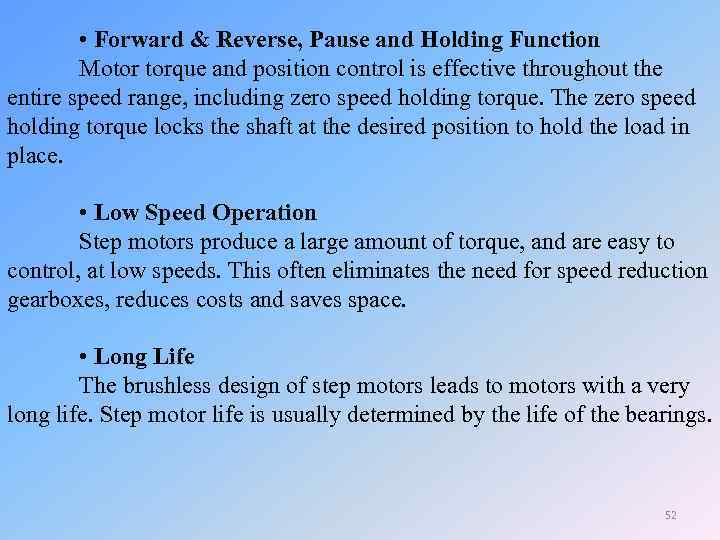• Forward & Reverse, Pause and Holding Function Motor torque and position control is effective throughout the entire speed range, including zero speed holding torque. The zero speed holding torque locks the shaft at the desired position to hold the load in place. • Low Speed Operation Step motors produce a large amount of torque, and are easy to control, at low speeds. This often eliminates the need for speed reduction gearboxes, reduces costs and saves space. • Long Life The brushless design of step motors leads to motors with a very long life. Step motor life is usually determined by the life of the bearings. 52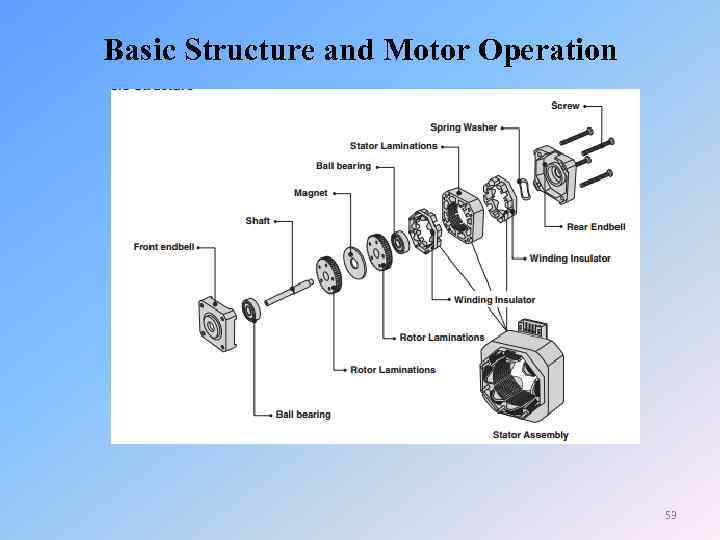Basic Structure and Motor Operation 53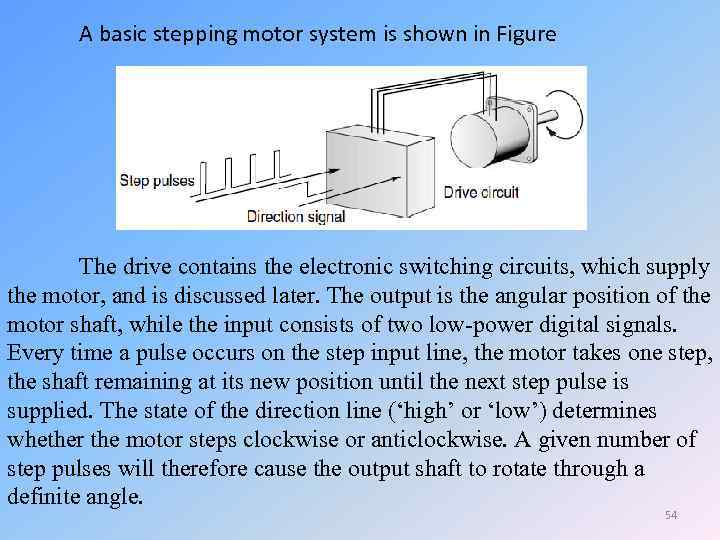A basic stepping motor system is shown in Figure The drive contains the electronic switching circuits, which supply the motor, and is discussed later. The output is the angular position of the motor shaft, while the input consists of two low-power digital signals. Every time a pulse occurs on the step input line, the motor takes one step, the shaft remaining at its new position until the next step pulse is supplied. The state of the direction line (‘high’ or ‘low’) determines whether the motor steps clockwise or anticlockwise. A given number of step pulses will therefore cause the output shaft to rotate through a definite angle. 54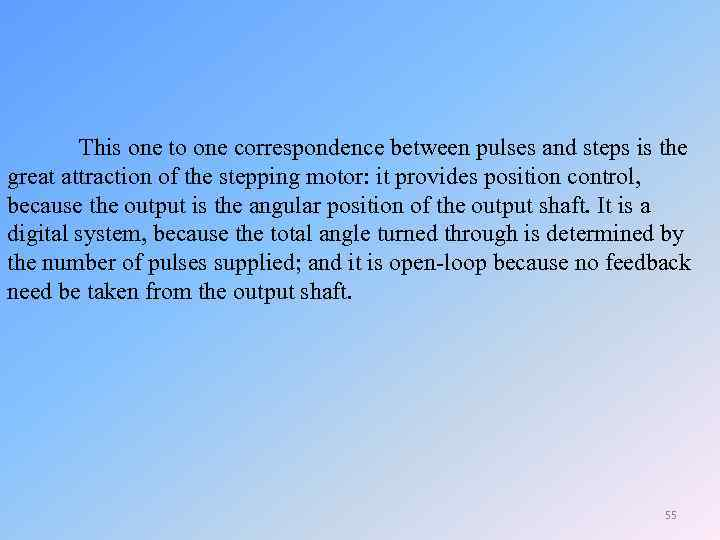This one to one correspondence between pulses and steps is the great attraction of the stepping motor: it provides position control, because the output is the angular position of the output shaft. It is a digital system, because the total angle turned through is determined by the number of pulses supplied; and it is open-loop because no feedback need be taken from the output shaft. 55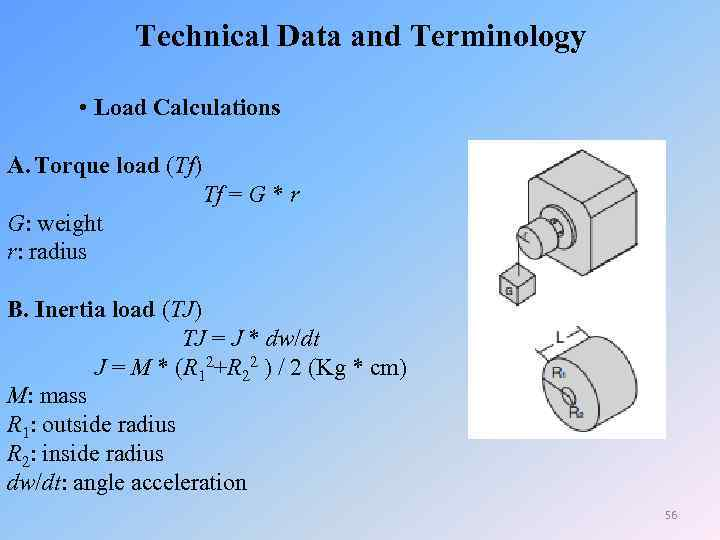Technical Data and Terminology • Load Calculations A. Torque load (Tf) Tf = G * r G: weight r: radius B. Inertia load (TJ) TJ = J * dw/dt J = M * (R 12+R 22 ) / 2 (Kg * cm) M: mass R 1: outside radius R 2: inside radius dw/dt: angle acceleration 56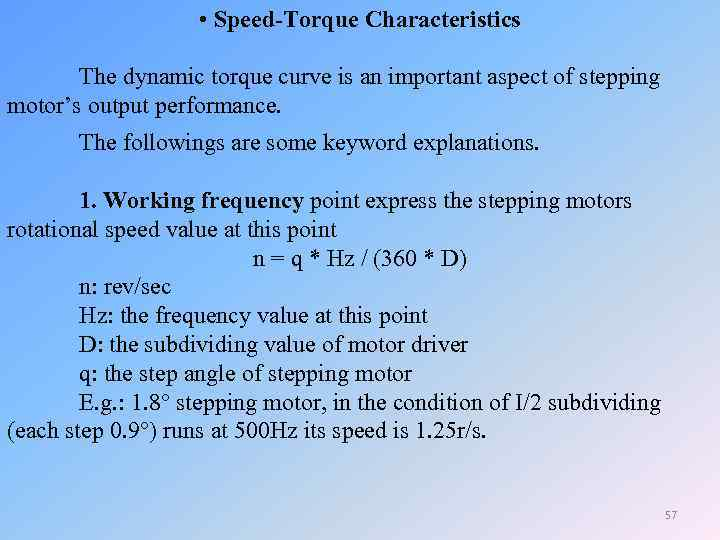• Speed-Torque Characteristics The dynamic torque curve is an important aspect of stepping motor’s output performance. The followings are some keyword explanations. 1. Working frequency point express the stepping motors rotational speed value at this point n = q * Hz / (360 * D) n: rev/sec Hz: the frequency value at this point D: the subdividing value of motor driver q: the step angle of stepping motor E. g. : 1. 8° stepping motor, in the condition of I/2 subdividing (each step 0. 9°) runs at 500 Hz its speed is 1. 25 r/s. 57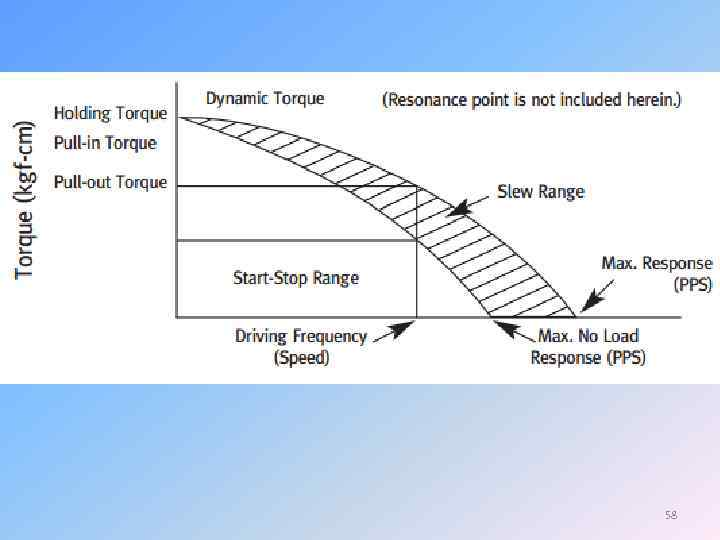58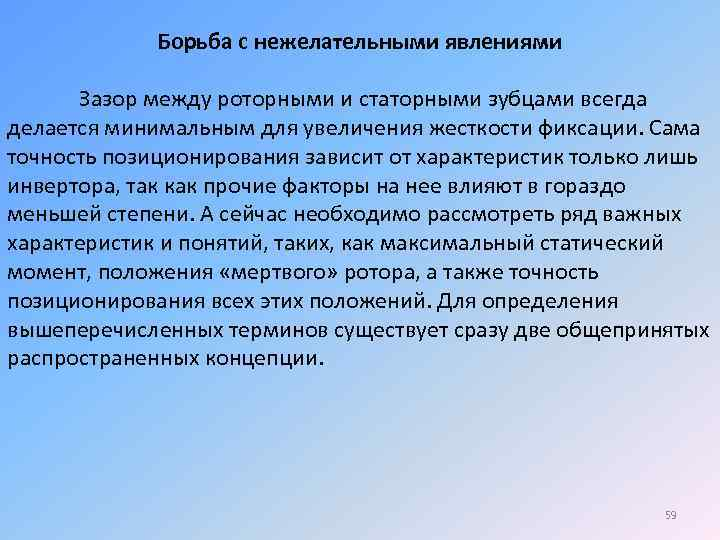Борьба с нежелательными явлениями Зазор между роторными и статорными зубцами всегда делается минимальным для увеличения жесткости фиксации. Сама точность позиционирования зависит от характеристик только лишь инвертора, так как прочие факторы на нее влияют в гораздо меньшей степени. А сейчас необходимо рассмотреть ряд важных характеристик и понятий, таких, как максимальный статический момент, положения «мертвого» ротора, а также точность позиционирования всех этих положений. Для определения вышеперечисленных терминов существует сразу две общепринятых распространенных концепции. 59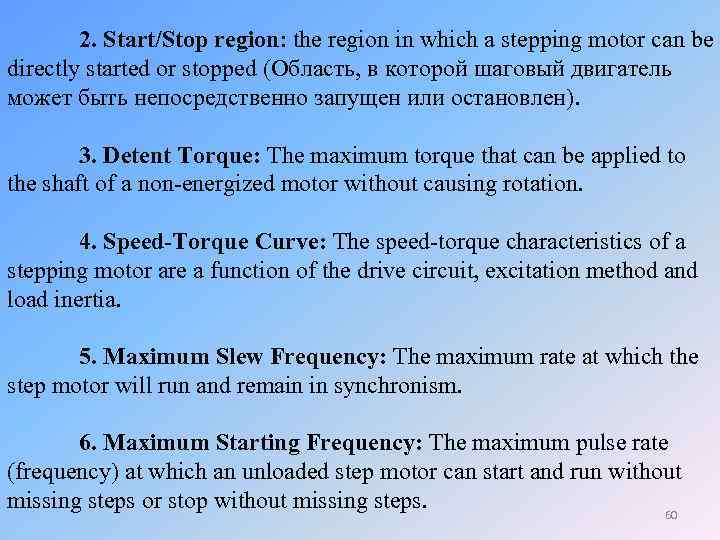2. Start/Stop region: the region in which a stepping motor can be directly started or stopped (Область, в которой шаговый двигатель может быть непосредственно запущен или остановлен). 3. Detent Torque: The maximum torque that can be applied to the shaft of a non-energized motor without causing rotation. 4. Speed-Torque Curve: The speed-torque characteristics of a stepping motor are a function of the drive circuit, excitation method and load inertia. 5. Maximum Slew Frequency: The maximum rate at which the step motor will run and remain in synchronism. 6. Maximum Starting Frequency: The maximum pulse rate (frequency) at which an unloaded step motor can start and run without missing steps or stop without missing steps. 60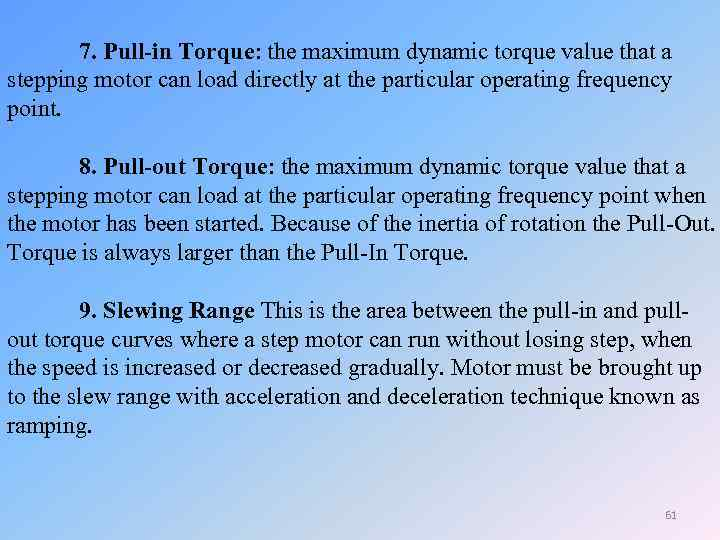7. Pull-in Torque: the maximum dynamic torque value that a stepping motor can load directly at the particular operating frequency point. 8. Pull-out Torque: the maximum dynamic torque value that a stepping motor can load at the particular operating frequency point when the motor has been started. Because of the inertia of rotation the Pull-Out. Torque is always larger than the Pull-In Torque. 9. Slewing Range This is the area between the pull-in and pullout torque curves where a step motor can run without losing step, when the speed is increased or decreased gradually. Motor must be brought up to the slew range with acceleration and deceleration technique known as ramping. 61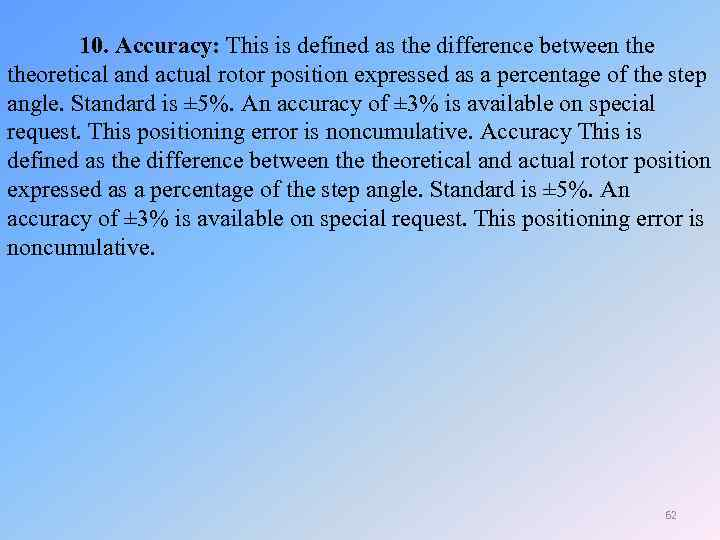10. Accuracy: This is defined as the difference between theoretical and actual rotor position expressed as a percentage of the step angle. Standard is ± 5%. An accuracy of ± 3% is available on special request. This positioning error is noncumulative. Accuracy This is defined as the difference between theoretical and actual rotor position expressed as a percentage of the step angle. Standard is ± 5%. An accuracy of ± 3% is available on special request. This positioning error is noncumulative. 62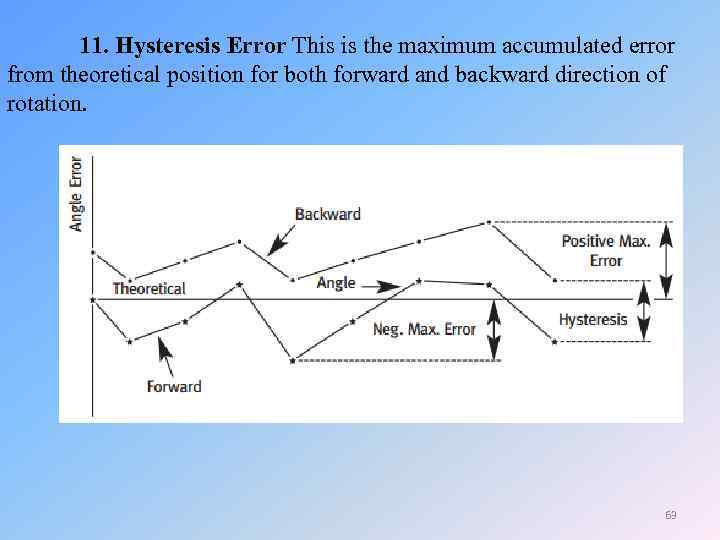11. Hysteresis Error This is the maximum accumulated error from theoretical position for both forward and backward direction of rotation. 63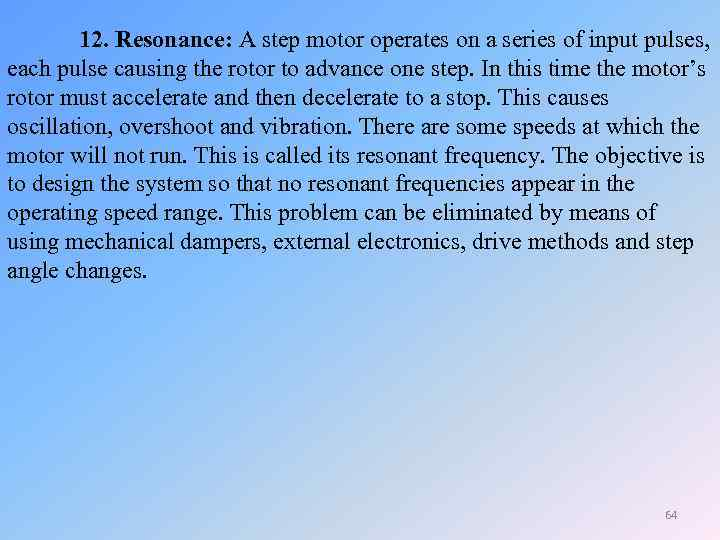12. Resonance: A step motor operates on a series of input pulses, each pulse causing the rotor to advance one step. In this time the motor’s rotor must accelerate and then decelerate to a stop. This causes oscillation, overshoot and vibration. There are some speeds at which the motor will not run. This is called its resonant frequency. The objective is to design the system so that no resonant frequencies appear in the operating speed range. This problem can be eliminated by means of using mechanical dampers, external electronics, drive methods and step angle changes. 64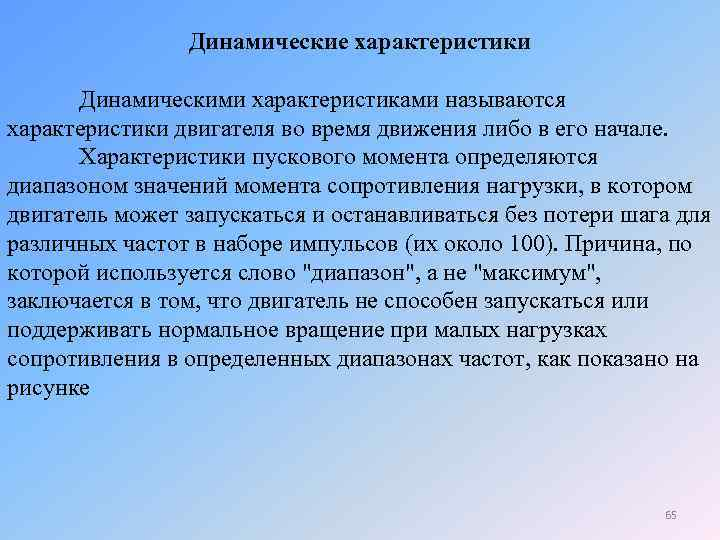Динамические характеристики Динамическими характеристиками называются характеристики двигателя во время движения либо в его начале. Характеристики пускового момента определяются диапазоном значений момента сопротивления нагрузки, в котором двигатель может запускаться и останавливаться без потери шага для различных частот в наборе импульсов (их около 100). Причина, по которой используется слово "диапазон", а не "максимум", заключается в том, что двигатель не способен запускаться или поддерживать нормальное вращение при малых нагрузках сопротивления в определенных диапазонах частот, как показано на рисунке 65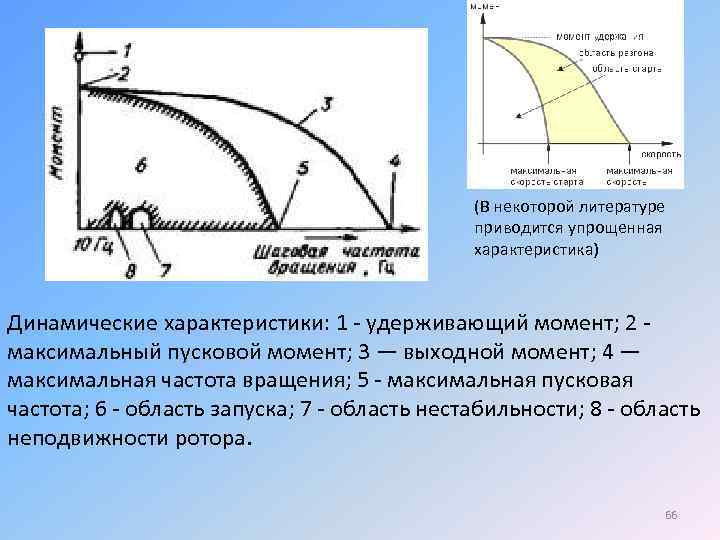(В некоторой литературе приводится упрощенная характеристика) Динамические характеристики: 1 - удерживающий момент; 2 максимальный пусковой момент; 3 — выходной момент; 4 — максимальная частота вращения; 5 - максимальная пусковая частота; 6 - область запуска; 7 - область нестабильности; 8 - область неподвижности ротора. 66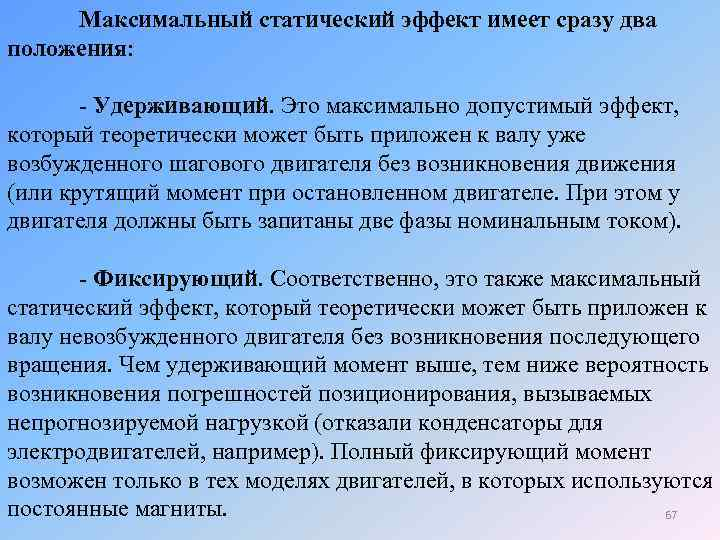Максимальный статический эффект имеет сразу два положения: - Удерживающий. Это максимально допустимый эффект, который теоретически может быть приложен к валу уже возбужденного шагового двигателя без возникновения движения (или крутящий момент при остановленном двигателе. При этом у двигателя должны быть запитаны две фазы номинальным током). - Фиксирующий. Соответственно, это также максимальный статический эффект, который теоретически может быть приложен к валу невозбужденного двигателя без возникновения последующего вращения. Чем удерживающий момент выше, тем ниже вероятность возникновения погрешностей позиционирования, вызываемых непрогнозируемой нагрузкой (отказали конденсаторы для электродвигателей, например). Полный фиксирующий момент возможен только в тех моделях двигателей, в которых используются постоянные магниты. 67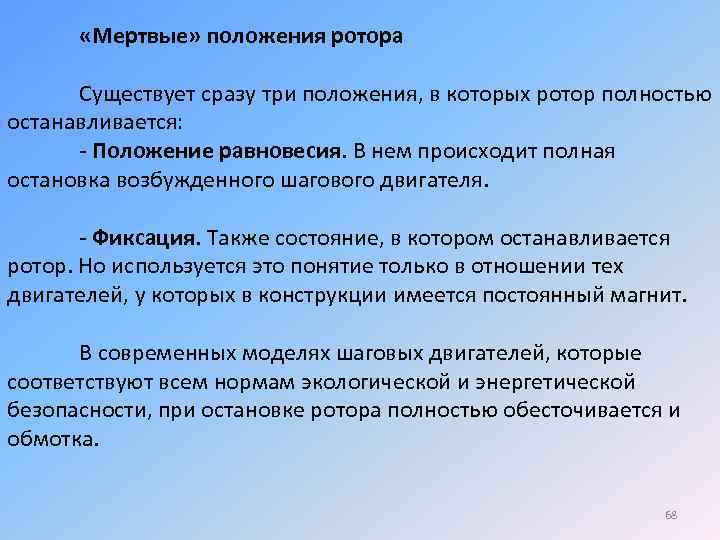«Мертвые» положения ротора Существует сразу три положения, в которых ротор полностью останавливается: - Положение равновесия. В нем происходит полная остановка возбужденного шагового двигателя. - Фиксация. Также состояние, в котором останавливается ротор. Но используется это понятие только в отношении тех двигателей, у которых в конструкции имеется постоянный магнит. В современных моделях шаговых двигателей, которые соответствуют всем нормам экологической и энергетической безопасности, при остановке ротора полностью обесточивается и обмотка. 68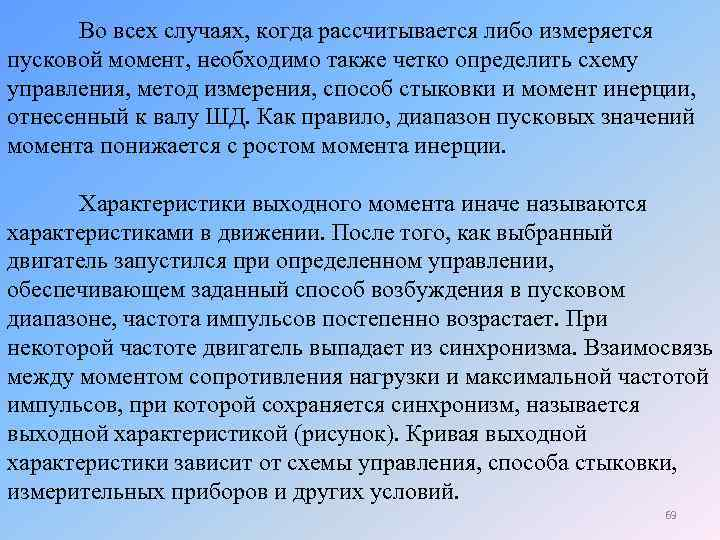Во всех случаях, когда рассчитывается либо измеряется пусковой момент, необходимо также четко определить схему управления, метод измерения, способ стыковки и момент инерции, отнесенный к валу ШД. Как правило, диапазон пусковых значений момента понижается с ростом момента инерции. Характеристики выходного момента иначе называются характеристиками в движении. После того, как выбранный двигатель запустился при определенном управлении, обеспечивающем заданный способ возбуждения в пусковом диапазоне, частота импульсов постепенно возрастает. При некоторой частоте двигатель выпадает из синхронизма. Взаимосвязь между моментом сопротивления нагрузки и максимальной частотой импульсов, при которой сохраняется синхронизм, называется выходной характеристикой (рисунок). Кривая выходной характеристики зависит от схемы управления, способа стыковки, измерительных приборов и других условий. 69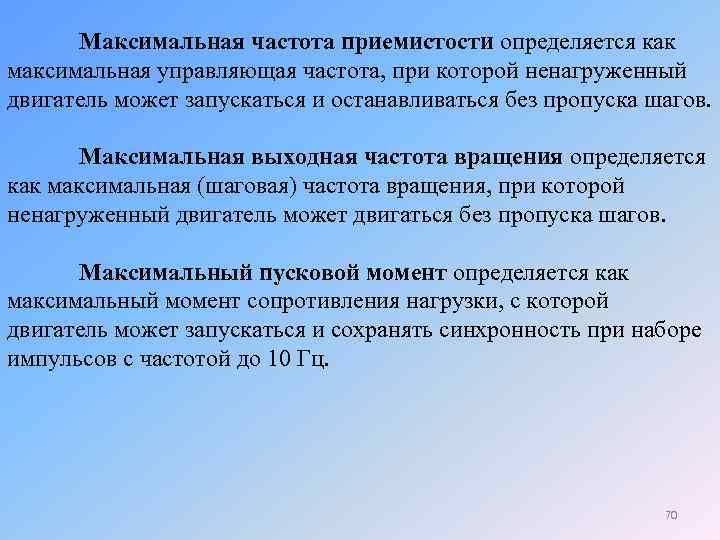Максимальная частота приемистости определяется как максимальная управляющая частота, при которой ненагруженный двигатель может запускаться и останавливаться без пропуска шагов. Максимальная выходная частота вращения определяется как максимальная (шаговая) частота вращения, при которой ненагруженный двигатель может двигаться без пропуска шагов. Максимальный пусковой момент определяется как максимальный момент сопротивления нагрузки, с которой двигатель может запускаться и сохранять синхронность при наборе импульсов с частотой до 10 Гц. 70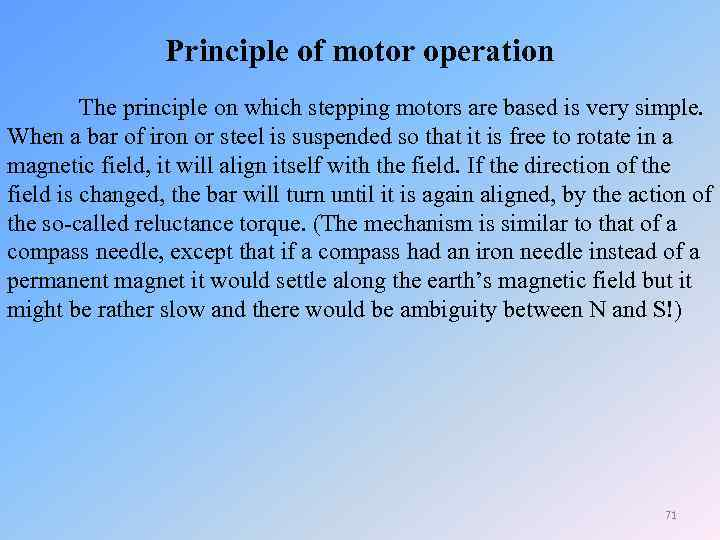Principle of motor operation The principle on which stepping motors are based is very simple. When a bar of iron or steel is suspended so that it is free to rotate in a magnetic field, it will align itself with the field. If the direction of the field is changed, the bar will turn until it is again aligned, by the action of the so-called reluctance torque. (The mechanism is similar to that of a compass needle, except that if a compass had an iron needle instead of a permanent magnet it would settle along the earth’s magnetic field but it might be rather slow and there would be ambiguity between N and S!) 71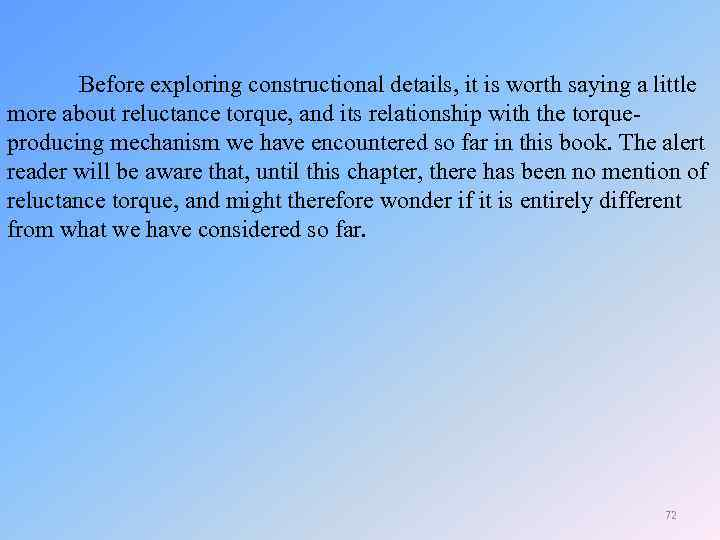Before exploring constructional details, it is worth saying a little more about reluctance torque, and its relationship with the torqueproducing mechanism we have encountered so far in this book. The alert reader will be aware that, until this chapter, there has been no mention of reluctance torque, and might therefore wonder if it is entirely different from what we have considered so far. 72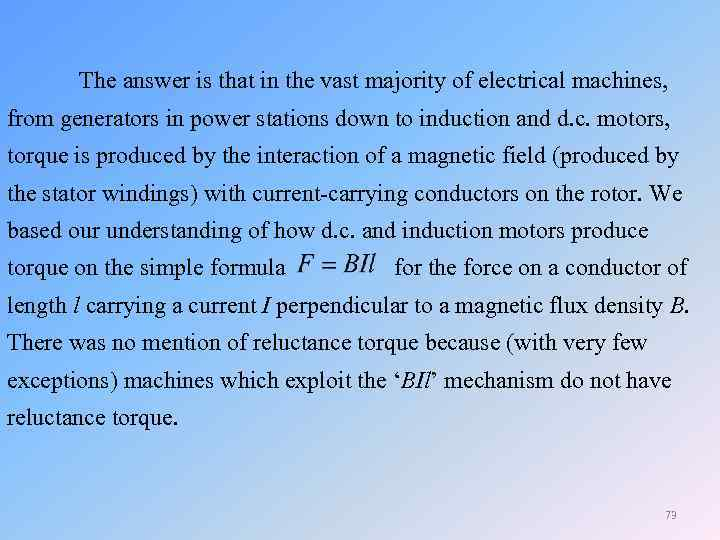The answer is that in the vast majority of electrical machines, from generators in power stations down to induction and d. c. motors, torque is produced by the interaction of a magnetic field (produced by the stator windings) with current-carrying conductors on the rotor. We based our understanding of how d. c. and induction motors produce torque on the simple formula for the force on a conductor of length l carrying a current I perpendicular to a magnetic flux density B. There was no mention of reluctance torque because (with very few exceptions) machines which exploit the ‘BIl’ mechanism do not have reluctance torque. 73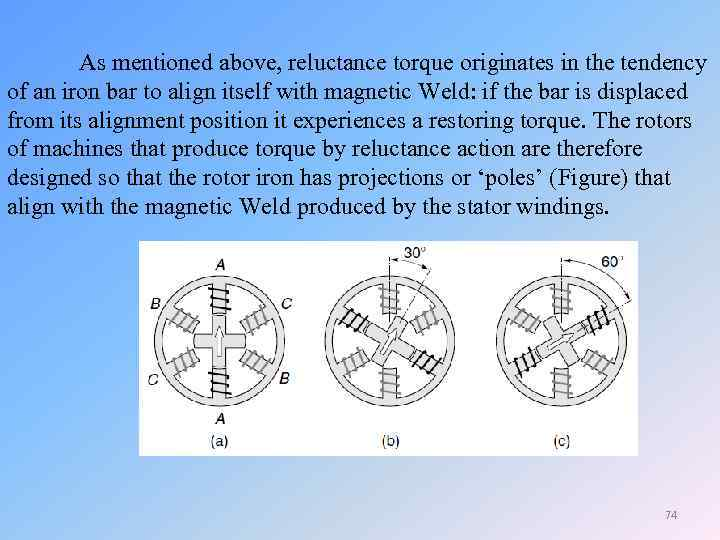As mentioned above, reluctance torque originates in the tendency of an iron bar to align itself with magnetic Weld: if the bar is displaced from its alignment position it experiences a restoring torque. The rotors of machines that produce torque by reluctance action are therefore designed so that the rotor iron has projections or ‘poles’ (Figure) that align with the magnetic Weld produced by the stator windings. 74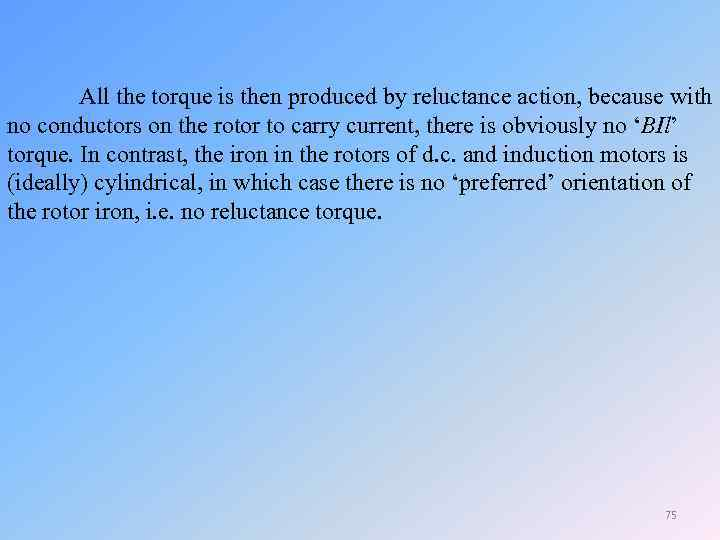All the torque is then produced by reluctance action, because with no conductors on the rotor to carry current, there is obviously no ‘BIl’ torque. In contrast, the iron in the rotors of d. c. and induction motors is (ideally) cylindrical, in which case there is no ‘preferred’ orientation of the rotor iron, i. e. no reluctance torque. 75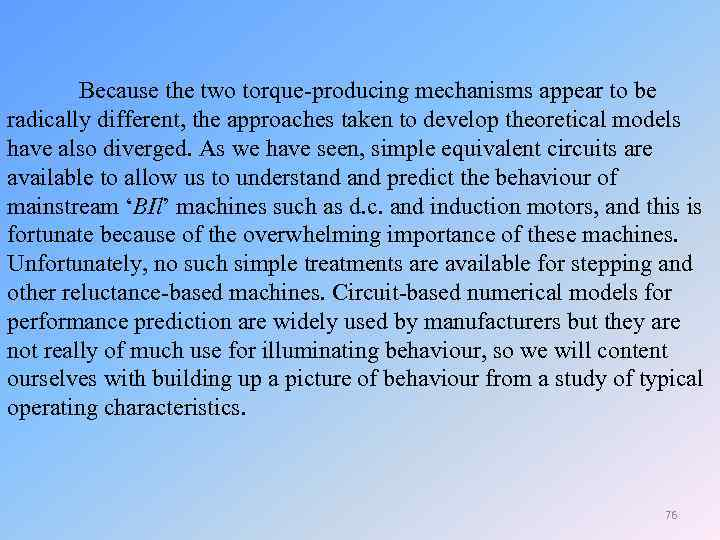Because the two torque-producing mechanisms appear to be radically different, the approaches taken to develop theoretical models have also diverged. As we have seen, simple equivalent circuits are available to allow us to understand predict the behaviour of mainstream ‘BIl’ machines such as d. c. and induction motors, and this is fortunate because of the overwhelming importance of these machines. Unfortunately, no such simple treatments are available for stepping and other reluctance-based machines. Circuit-based numerical models for performance prediction are widely used by manufacturers but they are not really of much use for illuminating behaviour, so we will content ourselves with building up a picture of behaviour from a study of typical operating characteristics. 76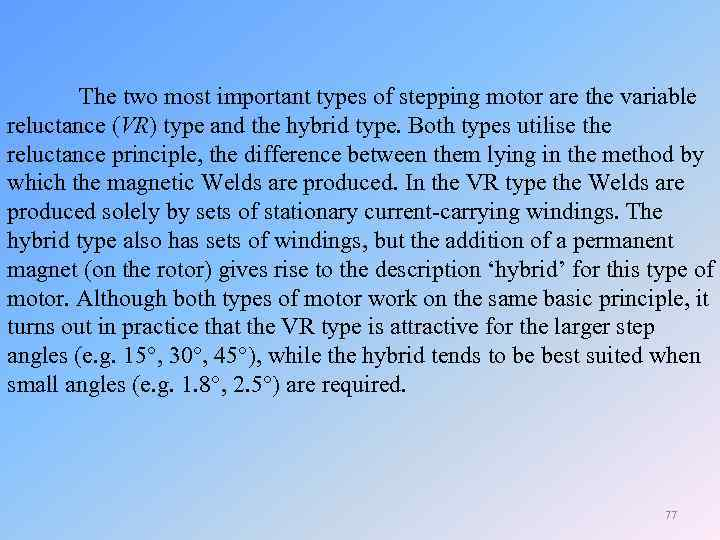The two most important types of stepping motor are the variable reluctance (VR) type and the hybrid type. Both types utilise the reluctance principle, the difference between them lying in the method by which the magnetic Welds are produced. In the VR type the Welds are produced solely by sets of stationary current-carrying windings. The hybrid type also has sets of windings, but the addition of a permanent magnet (on the rotor) gives rise to the description ‘hybrid’ for this type of motor. Although both types of motor work on the same basic principle, it turns out in practice that the VR type is attractive for the larger step angles (e. g. 15 , 30 , 45 ), while the hybrid tends to be best suited when small angles (e. g. 1. 8 , 2. 5 ) are required. 77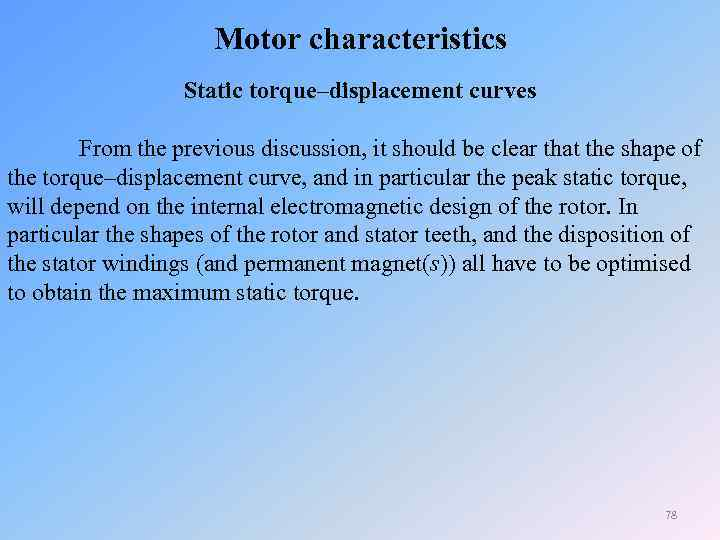Motor characteristics Static torque–displacement curves From the previous discussion, it should be clear that the shape of the torque–displacement curve, and in particular the peak static torque, will depend on the internal electromagnetic design of the rotor. In particular the shapes of the rotor and stator teeth, and the disposition of the stator windings (and permanent magnet(s)) all have to be optimised to obtain the maximum static torque. 78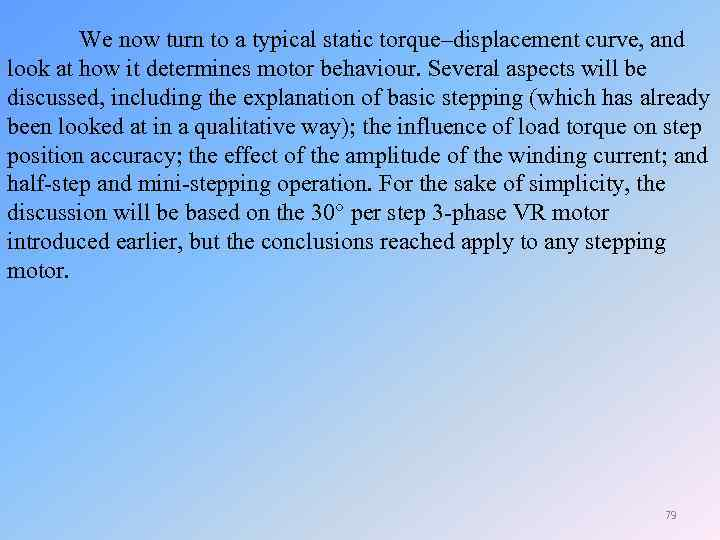We now turn to a typical static torque–displacement curve, and look at how it determines motor behaviour. Several aspects will be discussed, including the explanation of basic stepping (which has already been looked at in a qualitative way); the influence of load torque on step position accuracy; the effect of the amplitude of the winding current; and half-step and mini-stepping operation. For the sake of simplicity, the discussion will be based on the 30 per step 3 -phase VR motor introduced earlier, but the conclusions reached apply to any stepping motor. 79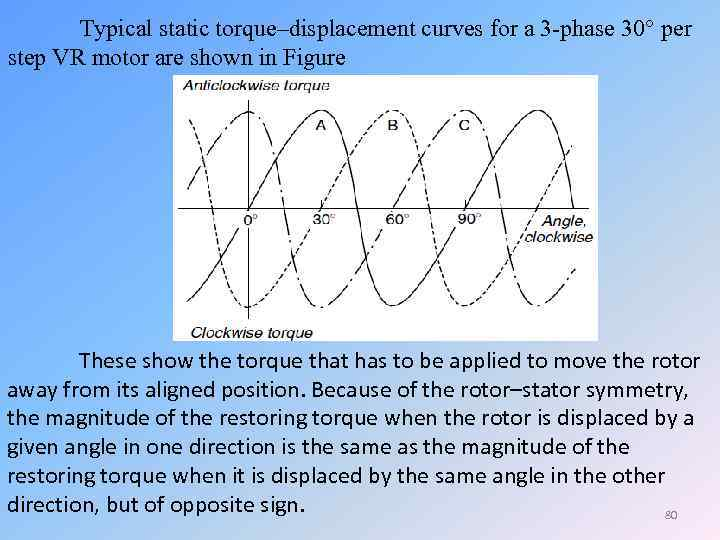Typical static torque–displacement curves for a 3 -phase 30 per step VR motor are shown in Figure These show the torque that has to be applied to move the rotor away from its aligned position. Because of the rotor–stator symmetry, the magnitude of the restoring torque when the rotor is displaced by a given angle in one direction is the same as the magnitude of the restoring torque when it is displaced by the same angle in the other direction, but of opposite sign. 80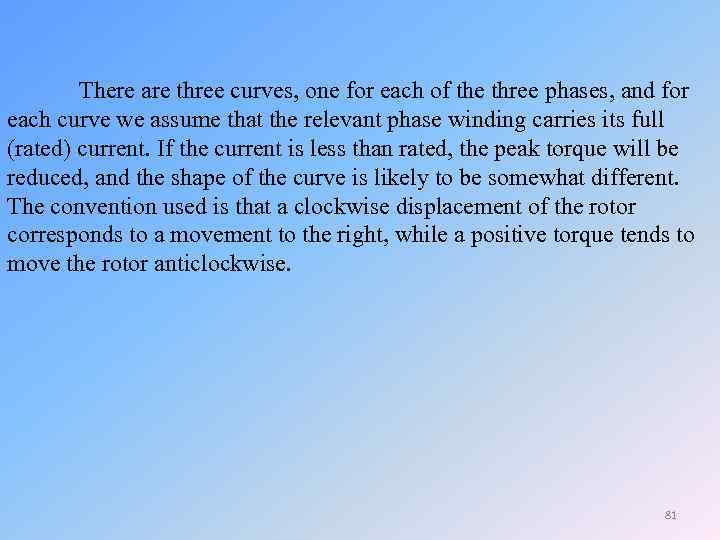There are three curves, one for each of the three phases, and for each curve we assume that the relevant phase winding carries its full (rated) current. If the current is less than rated, the peak torque will be reduced, and the shape of the curve is likely to be somewhat different. The convention used is that a clockwise displacement of the rotor corresponds to a movement to the right, while a positive torque tends to move the rotor anticlockwise. 81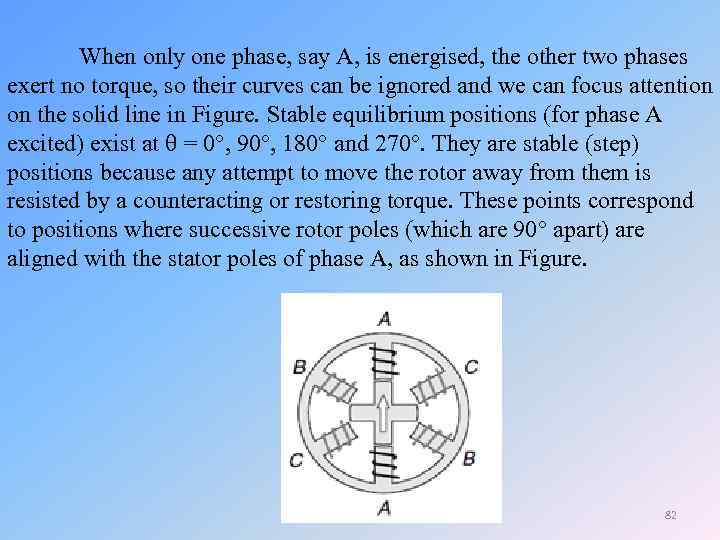When only one phase, say A, is energised, the other two phases exert no torque, so their curves can be ignored and we can focus attention on the solid line in Figure. Stable equilibrium positions (for phase A excited) exist at = 0 , 90 , 180 and 270. They are stable (step) positions because any attempt to move the rotor away from them is resisted by a counteracting or restoring torque. These points correspond to positions where successive rotor poles (which are 90 apart) are aligned with the stator poles of phase A, as shown in Figure. 82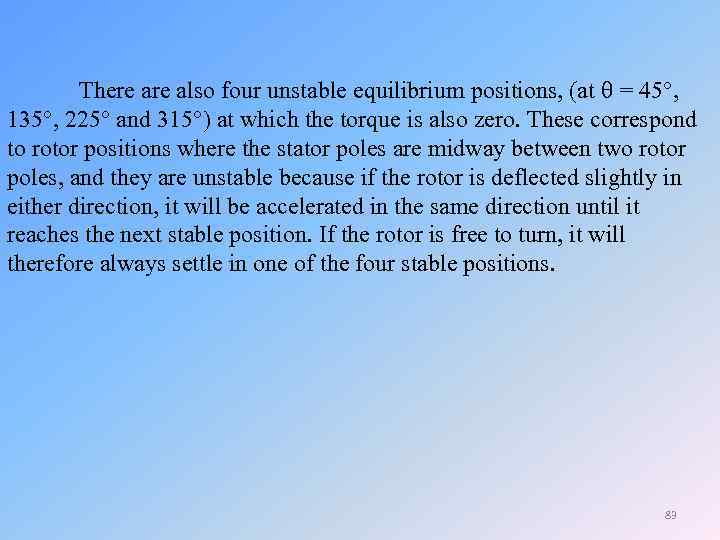There also four unstable equilibrium positions, (at = 45 , 135 , 225 and 315 ) at which the torque is also zero. These correspond to rotor positions where the stator poles are midway between two rotor poles, and they are unstable because if the rotor is deflected slightly in either direction, it will be accelerated in the same direction until it reaches the next stable position. If the rotor is free to turn, it will therefore always settle in one of the four stable positions. 83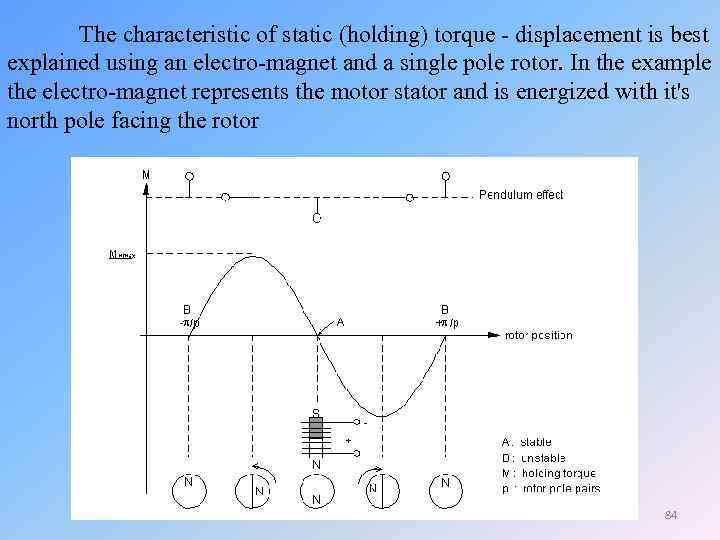The characteristic of static (holding) torque - displacement is best explained using an electro-magnet and a single pole rotor. In the example the electro-magnet represents the motor stator and is energized with it's north pole facing the rotor 84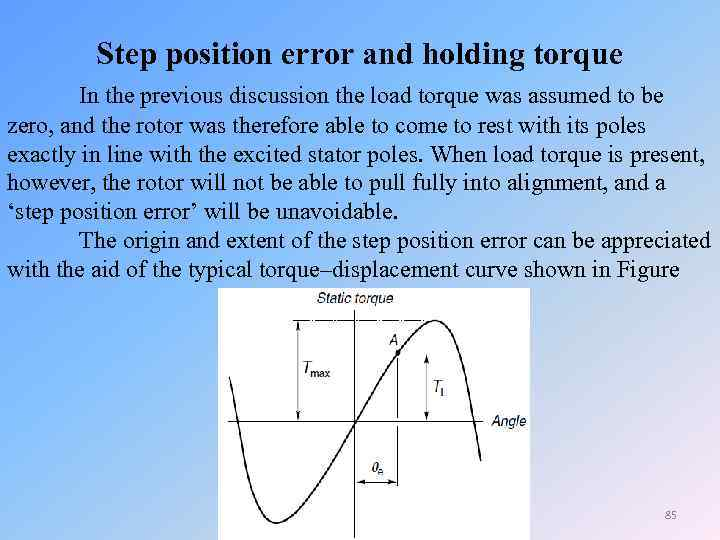Step position error and holding torque In the previous discussion the load torque was assumed to be zero, and the rotor was therefore able to come to rest with its poles exactly in line with the excited stator poles. When load torque is present, however, the rotor will not be able to pull fully into alignment, and a ‘step position error’ will be unavoidable. The origin and extent of the step position error can be appreciated with the aid of the typical torque–displacement curve shown in Figure 85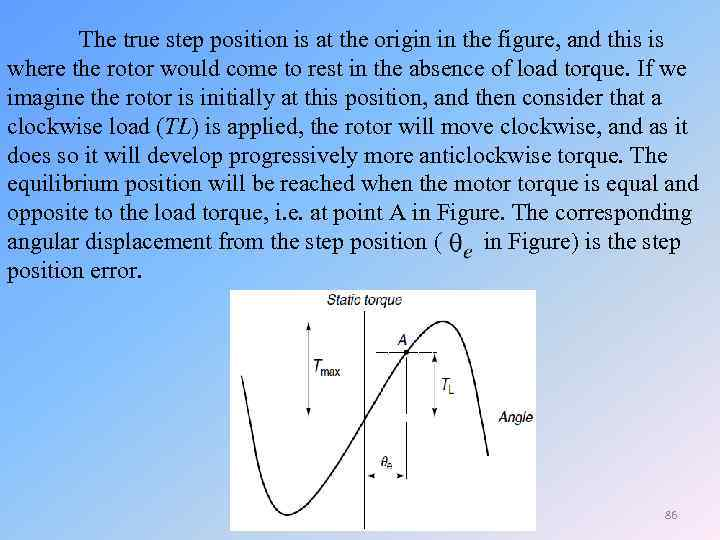The true step position is at the origin in the figure, and this is where the rotor would come to rest in the absence of load torque. If we imagine the rotor is initially at this position, and then consider that a clockwise load (TL) is applied, the rotor will move clockwise, and as it does so it will develop progressively more anticlockwise torque. The equilibrium position will be reached when the motor torque is equal and opposite to the load torque, i. e. at point A in Figure. The corresponding angular displacement from the step position ( in Figure) is the step position error. 86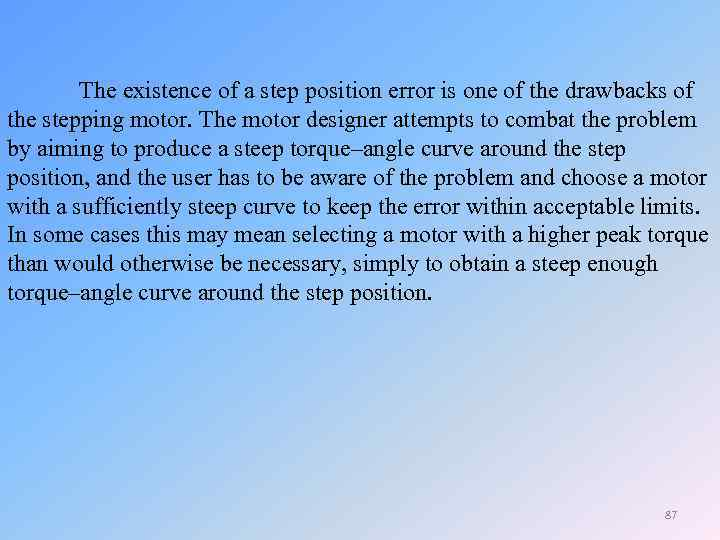The existence of a step position error is one of the drawbacks of the stepping motor. The motor designer attempts to combat the problem by aiming to produce a steep torque–angle curve around the step position, and the user has to be aware of the problem and choose a motor with a sufficiently steep curve to keep the error within acceptable limits. In some cases this may mean selecting a motor with a higher peak torque than would otherwise be necessary, simply to obtain a steep enough torque–angle curve around the step position. 87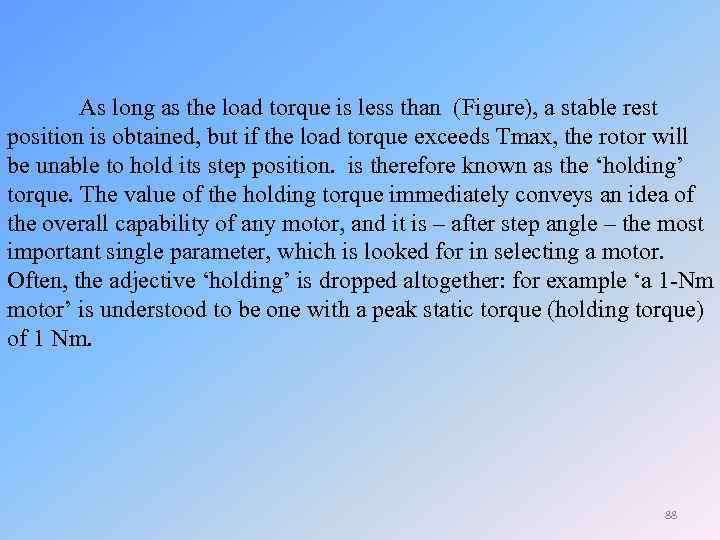As long as the load torque is less than (Figure), a stable rest position is obtained, but if the load torque exceeds Tmax, the rotor will be unable to hold its step position. is therefore known as the ‘holding’ torque. The value of the holding torque immediately conveys an idea of the overall capability of any motor, and it is – after step angle – the most important single parameter, which is looked for in selecting a motor. Often, the adjective ‘holding’ is dropped altogether: for example ‘a 1 -Nm motor’ is understood to be one with a peak static torque (holding torque) of 1 Nm. 88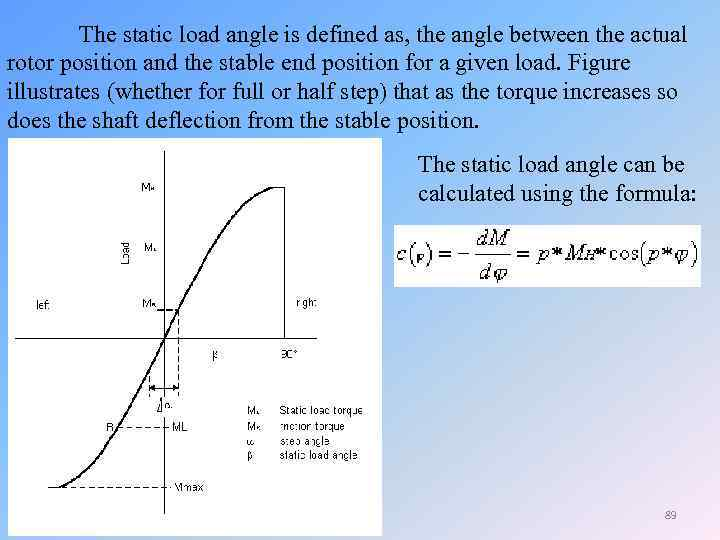The static load angle is defined as, the angle between the actual rotor position and the stable end position for a given load. Figure illustrates (whether for full or half step) that as the torque increases so does the shaft deflection from the stable position. The static load angle can be calculated using the formula: 89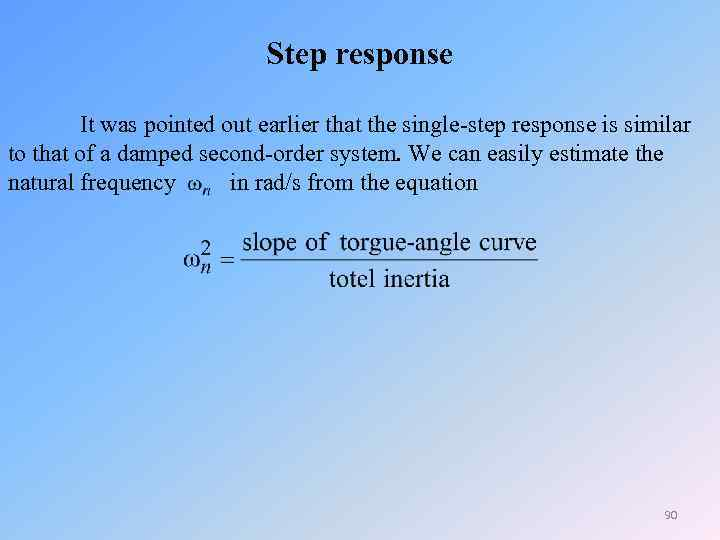Step response It was pointed out earlier that the single-step response is similar to that of a damped second-order system. We can easily estimate the natural frequency in rad/s from the equation 90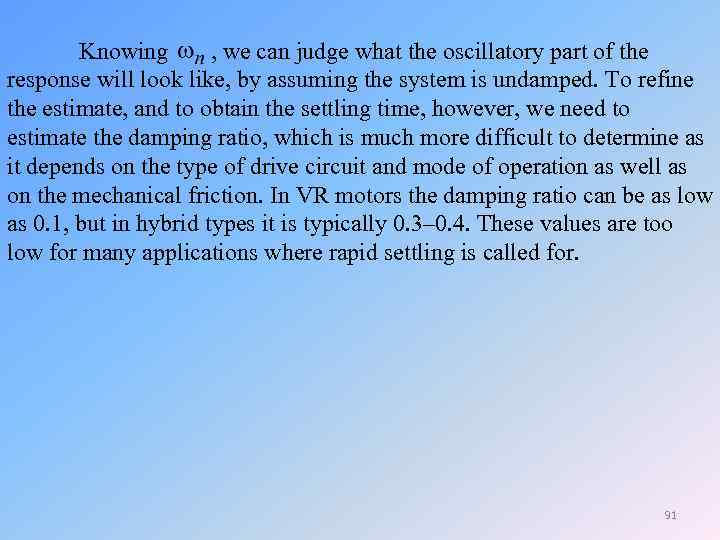Knowing , we can judge what the oscillatory part of the response will look like, by assuming the system is undamped. To refine the estimate, and to obtain the settling time, however, we need to estimate the damping ratio, which is much more difficult to determine as it depends on the type of drive circuit and mode of operation as well as on the mechanical friction. In VR motors the damping ratio can be as low as 0. 1, but in hybrid types it is typically 0. 3– 0. 4. These values are too low for many applications where rapid settling is called for. 91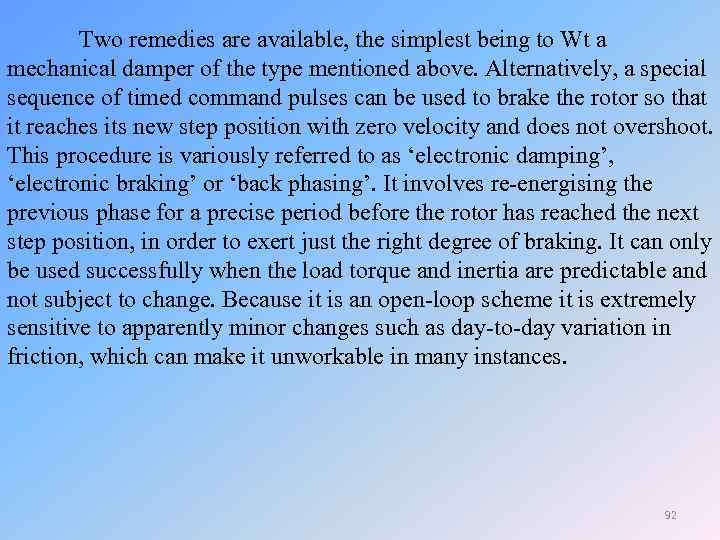Two remedies are available, the simplest being to Wt a mechanical damper of the type mentioned above. Alternatively, a special sequence of timed command pulses can be used to brake the rotor so that it reaches its new step position with zero velocity and does not overshoot. This procedure is variously referred to as ‘electronic damping’, ‘electronic braking’ or ‘back phasing’. It involves re-energising the previous phase for a precise period before the rotor has reached the next step position, in order to exert just the right degree of braking. It can only be used successfully when the load torque and inertia are predictable and not subject to change. Because it is an open-loop scheme it is extremely sensitive to apparently minor changes such as day-to-day variation in friction, which can make it unworkable in many instances. 92